This was a second test-fire of that radiography system, using yet-another board where I already know where everything is (on account of having designed it.)

This time, the victim is a FG TRNG Mainboard:

Click for full resolution (Warning: 16MB)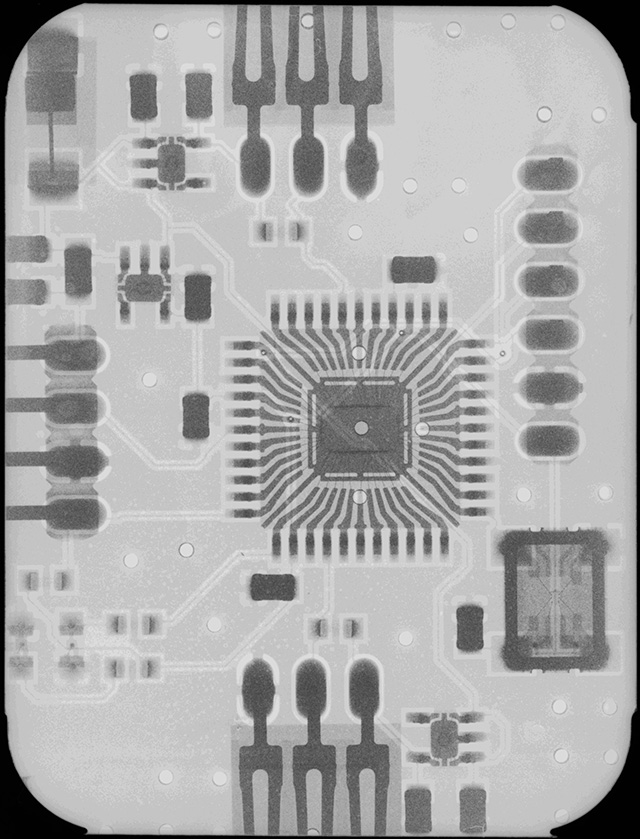For comparison, a visible-light photograph of the same section:

Detail of XC9572 CPLD:

Detail of 14.7456MHz quartz resonator:

Exposure: 100 sec. @ 32kV, 0.3mA @ 25cm; film: "Eco-30".

Below is the result of test-firing a newly-installed miniature PCB radiography system.

The victim is a standard FG TRNG Analogue Unit:

Click for full resolution (Warning: 14MB)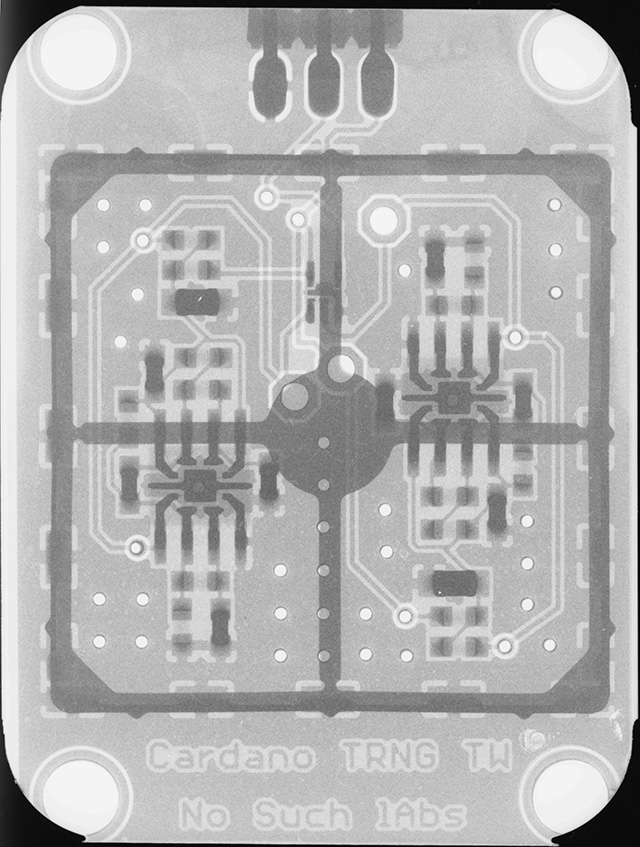Detail of left op amp, with bonding whiskers visible: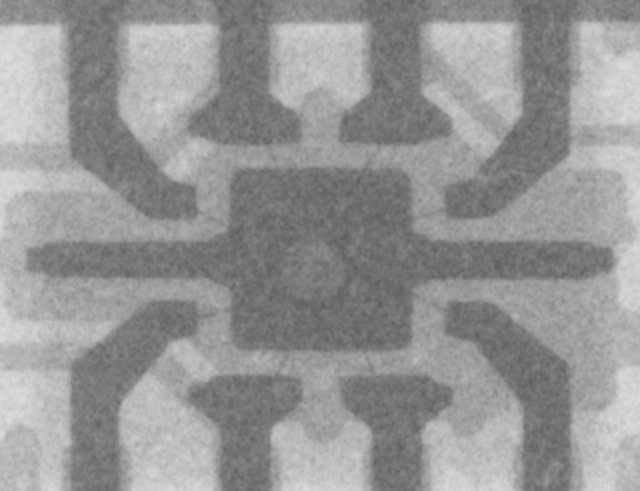The substrate, aluminum RF shield frame, SMT contacts, vias, and the two layers of the PCB are clearly distinguishable. Likewise resistors (nearly transparent) from capacitors (comparatively metal-heavy.)

Exposure: 100 sec. @ 32kV; 0.3mA @ 25cm. film: "Eco-30".

More interesting applications -- later.

## Lost Technology: The PQ3QI-01 Sunlight-Readable Display.

In the last quarter of the previous year, I got hold of a virginal (sealed OEM-crate) PQ3QI-01 sunlight-viewable LCD panel (and, after several unsuccessful attempts -- an old box where it fits.) These marvels are long out of print but apparently still available, somehow, from the Chinese, at around 100 \$ per.

If the backlight lamp is used, even on minimal power, the colour picture remains sharp in direct sunlight, which cannot be said for any other LCD which I have previously owned.

When the lamp is not used, the reflective backing of the crystal becomes visible and makes for a razor-sharp monochrome picture, if the contents of the display are appropriately configured.

I promised several people a photograph, which follows:

Lamp enabled, Emacs in typical working mode: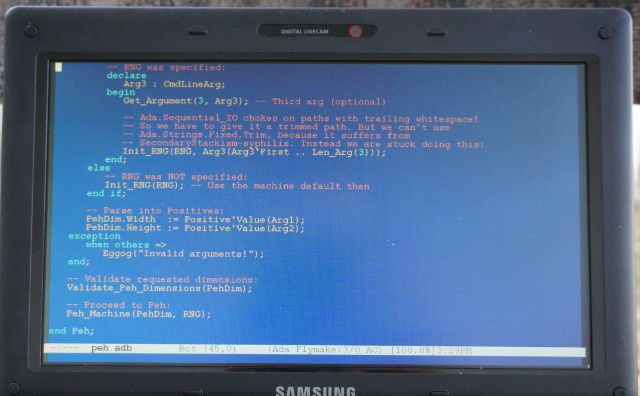Lamp disabled, and Emacs background set to white: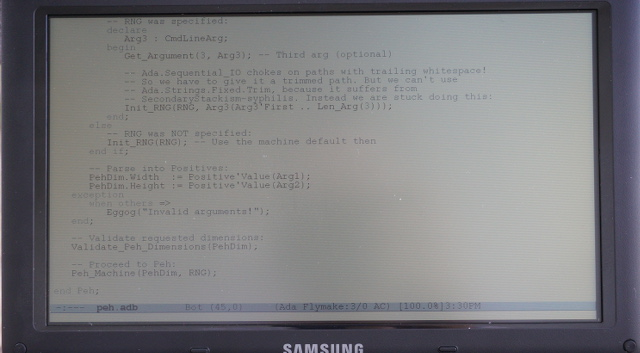The spot of shade is the fault of my head plus the camera. Possibly I will re-take this picture when winter ends, for a clearer portrait, the above does not really do the thing justice.

The machine (otherwise uninteresting, and costing around 50 \$ second-hand) was experimentally found to run, given a full charge, for 4.5 - 5 hours with the lamp running; and 7.5 - 8 without (given minimal CPU load.)

Before anyone asks, I have no idea why the manufacturer went broke. It was, IMHO, a great product, and in so far as I know had no competition whatsoever, nor is any direct replacement available today (aside from the Chinese old-stock.)

Posted in: Hardware, NonLoper, Photo by Stanislav 1 Comment

## “Finite Field Arithmetic.” Chapter 16A: The Miller-Rabin Test.

This article is part of a series of hands-on tutorials introducing FFA, or the Finite Field Arithmetic library. FFA differs from the typical "Open Sores" abomination, in that -- rather than trusting the author blindly with their lives -- prospective users are expected to read and fully understand every single line. In exactly the same manner that you would understand and pack your own parachute. The reader will assemble and test a working FFA with his own hands, and at the same time grasp the purpose of each moving part therein.

You will need:

Add the above vpatches and seals to your V-set, and press to ffa_ch16_miller_rabin.kv.vpatch.

You should end up with the same directory structure as previously.

As of Chapter 16, the versions of FFACalc and FFA are 253 and 253, respectively.

Now compile ffacalc:

```cd ffacalc gprbuild```

But do not run it quite yet.

First, the mail bag!

Reader bvt has given me to know that he has read and signed Chapters 1 - 6:

He also published a report of his interesting experiment with a variant of the Karatsuba multiplication algorithm.

Now, let's eat the meat of this Chapter.

We will add a very useful feature to FFA and FFACalc, a constant-spacetime Miller-Rabin Monte Carlo Primality Test:

Op Description # Ins # Outs Notes
P Perform a single shot of the Miller-Rabin Monte Carlo Primality Test on N, the second item on the stack, using the top item as the Witness parameter for the test. Places 1 on the stack if N was found to be composite; or 0, if N was not found to be composite. 2 1 If the supplied Witness does not satisfy the inequality 2 ≤ Witness ≤ N - 2 , it will be mapped via modular arithmetic to a value which satisfies it.
N ∈ {0, 1} will be pronounced composite under any Witness; N ∈ {2, 3} will be judged not composite under any Witness.
Any N which was found to be composite under any particular Witness, is in fact composite. The converse, is however, not true; see Ch. 16 discussion.

The proof of the Miller-Rabin method will be given in the next chapter, 16B.

Presently, the reader is invited to satisfy himself that the given program conforms to the stated variant of Miller-Rabin, and executes it in constant-spacetime:

Algorithm 1: Miller-Rabin Monte Carlo Primality Test.

For an odd integer N ≥ 5 and a positive integer Witness where 2 ≤ W ≤ N - 2:

1. Find the unique values R ≥ 1 and odd K such that 2R × K = N - 1.
2. T := WK mod N.
3. If T ∈ {1, N - 1}:
Return Possibly-Prime.
4. Iterate R - 1 times:
5.    T := T2 mod N
6.    If T = N - 1:
Return Possibly-Prime.
7. Return Composite.

The astute reader will immediately observe that Algorithm 1 is not suitable for use in FFA, as it does not execute in constant time, and does not correctly handle all possible values of N: N < 5 and even N do not meet the stated constraints.

Additionally, given as most practical uses of the Miller-Rabin method involve a sequence of invocations with a random Witness parameter, it is necessary to force a potentially out-of-range Witness into the expected range, with a minimal loss of entropy.

So, let's generalize the algorithm:

Algorithm 2: Generalized Miller-Rabin Monte Carlo Primality Test.

For
any integers N and W:

1. ProbPrime  := 0.

2. DegenComp  := {N ∈ {0, 1, even and ≠ 2} → 1, else 0}.

3. DegenPrime := {N ∈ {2, 3} → 1, else 0}.

4. BW        := W mod (N - 1).

5. If BW < 2:
BW := 2;
6. Find the unique values R ≥ 1 and odd K such that 2R × K = N - 1.
7. T := BWK mod N.
8. If T ∈ {1, N - 1}:
ProbPrime := 1.
9. Iterate R - 1 times:
10.    T := T2 mod N
11.    If T = N - 1:
ProbPrime := 1.
12. If DegenComp = 1:
Return Composite.
13. If DegenPrime = 1 or ProbPrime = 1:
Return Possibly-Prime.
14. Return Composite.

Still not a constant-time algorithm; but the sharp reader will be able to identify the missing ingredients.

Now let's rewrite it into a form suitable for use in FFA:

Algorithm 3: Constant-Time Generalized Miller-Rabin Monte Carlo Primality Test.

For
any integers N and W:

1. DegenComp  := (N = 0) OR (N = 1) OR ((N ≠ 2) AND (N mod 2 = 0))

2. DegenPrime := (N = 2) OR (N = 3)

3. ProbPrime := DegenPrime.

4. BW        := W mod (N - 1).

5. BW        := Mux( 2 ← (BW < 2) , BW ← (BW ≥ 2) )
6. R         := Count_Bottom_Zeros(N).
7. K         := N >> R.
8. T         := BWK mod N.
9. ProbPrime := ProbPrime OR (T = 1) OR (T = N - 1)
10. I         := 1
11. Iterate FZ_Bitness(N) - 1 times:
12.    T         := T2 mod N
13.    ProbPrime := ProbPrime OR ((I < R) AND (T = N - 1))
14.    I         := I + 1
15. Return DegenComp OR (1 - ProbPrime).
16. Returned value 0 corresponds to Possibly-Prime; 1 to Composite.

This variant will carry out the Miller-Rabin test on any possible candidate integer of a given FFA Bitness, without leaking the integer or the Witness via timing side-channel, and the Witness will be forced into the valid range. To my knowledge, this is the first publication of an algorithm which has these characteristics.

Without further delay, let's implement Algorithm 3 as a FFA subroutine:

```package body FZ_Prime is   -- Find the highest power of 2 which divides N. ( or 0 if N is zero. ) function FZ_Count_Bottom_Zeros(N : in FZ) return FZBit_Index is   -- The result (default : 0, will remain 0 if N is in fact zero) Index : Word := 0;   -- The currently-examined Word, starting from the highest Ni : Word;   -- The most recently-seen nonzero Word, if indeed any exist W : Word := 0;   -- 1 if currently-examined Word is zero, otherwise 0 NiZ : WBool;   begin   -- Find lowest non-zero Word (or simply stop at lowest, if N = 0) for i in reverse 0 .. Indices(N'Length - 1) loop Ni := N(N'First + i); -- Ni := current Word; NiZ := W_ZeroP(Ni); -- ... is this Word = 0? Index := W_Mux(Word(i), Index, NiZ); -- If NO, save its Index, W := W_Mux(Ni, W, NiZ); -- ... and save the Word. end loop;   -- Set Index to be the bit-position of the lowest non-zero Word: Index := Shift_Left(Index, BitnessLog2); -- Index := Index * Bitness   -- Handle degenerate case: if W is equal to 0, Index is not changed; -- Otherwise, start by advancing Index by an entire Word Bitness: Index := Index + ((0 - W_NZeroP(W)) and Word(Bitness));   -- Now crank back the Index by the number of high bits of W (i.e. -- starting from its top) that must be discarded for W to become zero: for b in 1 .. Bitness loop   -- If W is non-zero at this time, decrement the Index: Index := Index - W_NZeroP(W);   -- Advance W left, i.e. discard the top bit of it: W := Shift_Left(W, 1);   end loop;   -- If N = 0, result will be 0; otherwise: length of bottom zeros in N. return FZBit_Index(Index);   end FZ_Count_Bottom_Zeros;     -- Constant-Time Miller-Rabin Test on N using the given Witness. -- Witness will be used as-is if it conforms to the valid bounds, -- i.e. 2 < = Witness <= N - 2; otherwise will be transformed into a -- valid Witness via modular arithmetic. -- Outputs ONE if N WAS FOUND composite; ZERO if NOT FOUND. -- Handles degenerate cases of N that M-R per se cannot eat: -- N=0, N=1: ALWAYS 'FOUND COMPOSITE'; 2, 3 - ALWAYS 'NOT FOUND'. -- If N is Even and not equal to 2, N is ALWAYS 'FOUND COMPOSITE.' -- For ALL other N, the output is equal to that of the M-R test. function FZ_MR_Composite_On_Witness(N : in FZ; Witness : in FZ) return WBool is   -- Widths of N, Witness, and Result are equal : subtype Width is Word_Index range N'Range;   -- Whether N is 'small' (i.e. 1 Word) and therefore possibly degenerate : N_Is_Small : constant WBool := FZ_OneWordP(N);   -- Head of N, for detecting (and handling) the degenerate-N case : N_Head : constant Word := FZ_Get_Head(N);   -- Zero and One are defined as COMPOSITE degenerate cases of N : N_Is_Zero_Or_One : constant WBool := N_Is_Small and (W_EqP(N_Head, 0) or W_EqP(N_Head, 1));   -- Two and Three are PRIME degenerate cases of N : N_Is_Two : constant WBool := N_Is_Small and W_EqP(N_Head, 2); N_Is_Three : constant WBool := N_Is_Small and W_EqP(N_Head, 3);   -- The COMPOSITE degenerate case of N where N != 2 and N is Even : N_Ne_2_Is_Even : constant WBool := (1 - N_Is_Two) and (1 - W_OddP(N_Head));   -- Degeneracy latch. If N is Zero or One, then set immediately : Degen_Composite : constant WBool := N_Is_Zero_Or_One or N_Ne_2_Is_Even;   -- Possible-primality latch. If N is Two or Three, then set immediately : Possibly_Prime : WBool := N_Is_Two or N_Is_Three;   -- The writable copy of N that we will operate on : X : FZ(Width) := N;   -- Potentially-replaced head of X (if degenerate N) : X_Head : Word := N_Head;   -- Will be Barrettoid(X), for operations modulo X : XBar : Barretoid(ZXMLength => X'Length + 1, BarretoidLength => 2 * X'Length);   -- The Bound Witness, constrained to valid range 2 < = BW <= X - 2 : BW : FZ(Width);   -- Head of BW, for detecting crossing of the lower bound : BW_Head : Word;   -- X - 1 (for M-R proper, and to constrain BW) : X_Minus_One : FZ(Width);   -- X - 1 = 2^R * K, with K odd : K : FZ(Width); R : FZBit_Index;   -- Working register for all M-R modular operations : T : FZ(Width);   -- For subtraction where no underflow can happen, barring cosmic ray: NoCarry : WZeroOrDie := 0;   begin   -- First: X, which will remain equal to N unless N is degenerate:   -- If N is one of the two prohibited small primes (2,3) X will become 5: X_Head := W_Mux(A => X_Head, B => 5, Sel => Possibly_Prime);   -- If one of the two prohibited small composites (0,1), or even, then 9: X_Head := W_Mux(A => X_Head, B => 9, Sel => Degen_Composite);   -- Given as we're stuck carrying out M-R even if N is degenerate case, -- then let the M-R do The Right Thing, for added cosmic ray resistance.   -- X gets a new head, if N was a degenerate case; else keeps old head: FZ_Set_Head(X, X_Head);   -- Compute X - 1. As now X > 3, underflow is not permitted: FZ_Sub_W(X => X, W => 1, Difference => X_Minus_One, Underflow => NoCarry);   -- Now, compute BW (Bound Witness), which satisfies 2 < = BW <= X - 2:   -- First, BW := Witness mod (X - 1). After this, 0 <= BW <= X - 2: FZ_Mod(Dividend => Witness, Divisor => X_Minus_One, Remainder => BW);   -- Now, adjust BW if it is found to be below Two (the lower bound) :   -- Get head of BW: BW_Head := FZ_Get_Head(BW);   -- If BW is equal to Zero or One, it will be forced to equal Two: BW_Head := W_Mux(A => BW_Head, B => 2, Sel => FZ_OneWordP(BW) and (W_EqP(BW_Head, 0) or W_EqP(BW_Head, 1)));   -- BW gets the new head, if it must; otherwise keeps its old head: FZ_Set_Head(BW, BW_Head);   -- We finished adjusting X and BW for degeneracies, and can now M-R:   -- Generate Barrettoid(X) to use in all of the modulo-X operations: FZ_Make_Barrettoid(Modulus => X, Result => XBar);   -- Find R >= 1, and odd K, where X − 1 = 2^R * K :   -- ... first, find R, the largest power of two which divides X - 1 : R := FZ_Count_Bottom_Zeros(X_Minus_One);   -- ... and now obtain K := X / 2^R, i.e., K := X >> R : FZ_Quiet_ShiftRight(N => X_Minus_One, ShiftedN => K, Count => R);   -- T := BW^K mod X FZ_Mod_Exp_Barrett(Base => BW, Exponent => K, Bar => XBar, Result => T);   -- if T = 1 or T = X - 1, then X is possibly-prime: Possibly_Prime := Possibly_Prime or FZ_EqP_W(T, 1) or FZ_EqP(T, X_Minus_One);   -- Needs R - 1 times, but perform for max possible count (gated): for i in 1 .. FZ_Bitness(N) - 1 loop   -- T := T^2 mod X FZ_Mod_Sqr_Barrett(X => T, Bar => XBar, Product => T);   -- if T = X - 1, and i < R, then X is possibly-prime: Possibly_Prime := Possibly_Prime or (FZ_EqP(T, X_Minus_One) and W_LtP(Word(i), Word(R)));   end loop;   -- The output, which will be 1 iff X WAS FOUND to be composite via BW, -- ... or if X was found to equal Zero or One (without regard to BW.) return (1 - Possibly_Prime) or Degen_Composite; -- If X was found to equal Two or Three, output will be 0 (under any BW).   end FZ_MR_Composite_On_Witness;   end FZ_Prime;```

Observe that degenerate values of N result in a re-assignment, such that the M-R procedure (which is carried out regardless of degeneracy, for constant-time operation) is forced to arrive at a correct output, acting as "anti-cosmicray" backup to the degeneracy latches.

Now, let's run the program, and get an idea of how it works. Let's create a FFA Tape which executes M-R, via random witnesses, on the first 12 Mersenne Primes:

```.2 .1`LS.1-?P[MR(2^]`#[- 1)=]{[Composite ]}{[Prime ]}Z   .3 .1`LS.1-?P[MR(2^]`#[- 1)=]{[Composite ]}{[Prime ]}Z   .5 .1`LS.1-?P[MR(2^]`#[- 1)=]{[Composite ]}{[Prime ]}Z   .7 .1`LS.1-?P[MR(2^]`#[- 1)=]{[Composite ]}{[Prime ]}Z   .d .1`LS.1-?P[MR(2^]`#[- 1)=]{[Composite ]}{[Prime ]}Z   .11 .1`LS.1-?P[MR(2^]`#[- 1)=]{[Composite ]}{[Prime ]}Z   .13 .1`LS.1-?P[MR(2^]`#[- 1)=]{[Composite ]}{[Prime ]}Z   .1f .1`LS.1-?P[MR(2^]`#[- 1)=]{[Composite ]}{[Prime ]}Z   .3d .1`LS.1-?P[MR(2^]`#[- 1)=]{[Composite ]}{[Prime ]}Z   .59 .1`LS.1-?P[MR(2^]`#[- 1)=]{[Composite ]}{[Prime ]}Z   .6b .1`LS.1-?P[MR(2^]`#[- 1)=]{[Composite ]}{[Prime ]}Z   .7f .1`LS.1-?P[MR(2^]`#[- 1)=]{[Composite ]}{[Prime ]}Z```

Now, run the tape as follows:

`\$ cat 12_mersenne.tape | ./bin/ffa_calc 256 3`

... and if successful, the output will be:

```MR(2^0000000000000000000000000000000000000000000000000000000000000002 - 1)=Prime MR(2^0000000000000000000000000000000000000000000000000000000000000003 - 1)=Prime MR(2^0000000000000000000000000000000000000000000000000000000000000005 - 1)=Prime MR(2^0000000000000000000000000000000000000000000000000000000000000007 - 1)=Prime MR(2^000000000000000000000000000000000000000000000000000000000000000D - 1)=Prime MR(2^0000000000000000000000000000000000000000000000000000000000000011 - 1)=Prime MR(2^0000000000000000000000000000000000000000000000000000000000000013 - 1)=Prime MR(2^000000000000000000000000000000000000000000000000000000000000001F - 1)=Prime MR(2^000000000000000000000000000000000000000000000000000000000000003D - 1)=Prime MR(2^0000000000000000000000000000000000000000000000000000000000000059 - 1)=Prime MR(2^000000000000000000000000000000000000000000000000000000000000006B - 1)=Prime MR(2^000000000000000000000000000000000000000000000000000000000000007F - 1)=Prime```

M-R is, of course, not the optimal means of confirming the primality of a suspected Mersenne prime. But it does give us a basic test: regardless of the output of your RNG, you will always see the above output.

Let's now try a large number known to be prime, the 18th Mersenne prime, 23217 - 1:

```.c91 .1`LS.1-?P[MR(2^]`#[- 1)=]{[Composite ]}{[Prime ]}Z```

Run this tape as follows:

`\$ cat 18th_mersenne.tape | ./bin/ffa_calc 4096 3`

... and the output will always be:

```MR(2^ 000000000000000000000000000000000000000000000000000000000000000000000000000000 000000000000000000000000000000000000000000000000000000000000000000000000000000 000000000000000000000000000000000000000000000000000000000000000000000000000000 000000000000000000000000000000000000000000000000000000000000000000000000000000 000000000000000000000000000000000000000000000000000000000000000000000000000000 000000000000000000000000000000000000000000000000000000000000000000000000000000 000000000000000000000000000000000000000000000000000000000000000000000000000000 000000000000000000000000000000000000000000000000000000000000000000000000000000 000000000000000000000000000000000000000000000000000000000000000000000000000000 000000000000000000000000000000000000000000000000000000000000000000000000000000 000000000000000000000000000000000000000000000000000000000000000000000000000000 000000000000000000000000000000000000000000000000000000000000000000000000000000 000000000000000000000000000000000000000000000000000000000000000000000000000000 0000000C91 - 1)=Prime```

Now, let's test the M-R mechanism on more cryptographically-typical inputs. Let's take one of the braindamaged RSA moduli from the Phuctor zoo (the stars of the SecLab incident), and a Witness of 3:

```.c08b0693f9ae0854829cd88d6538756df69ff8067d1556678f7e45b17437014374174c4 aca94bf1f83640928832b398f88c935c6a08177c4cbaa8b85002fee95068bd68487f286f e3b814d92d6147b3d90fba606701f72e1f205c3e06dba55f5e180e45c2225a6ca2061d2d 638ef42609c5d8225620107519628b35983e92e0930ff2e2b8a3a0d9da57a4f50aaefe21 c0b02f8a91587f3ea2337df593f2faea40cb0d6359fee2df45b14b4e8f20988c542b81c7 862f74ea3a3761c22f6ecef64efb2014ccdcf13fb251ed3160ee20f392d0a2200db105c4 5bc12badbaa53a00a1371a77e12de455824c10dafd87f9c150f1e3fb622a8bb68134764a 77a939371bbe63ede53591d1b2bf35ff2f15776a2e1670c8c0006973782c52e97ded5ad1 e4cc96cc4bffd73061e14059aa40dbbc89d46ea1e20500a0e5ac7ac374c277e8d745dc45 449505d1c1bafecd9df8aa75096fffe4cd2f164e2a12d35000782dd73a5b58f8064ea4c0 afe2066f31d336fe65c50a9dff8e3db8a379b182e6d440cb8903fad5ed8477bde7ac2c13 1a7cd47d94630e92f98f68b86d6288607d1ef03880ca18f4176caf08869df93e93433a08 20af7e82e5eed7fd39a2480d98c34f5862dd7ceb4f8382a84acad97d1ee8da685d2e4aa5 f26167a3385f0a3412e168162916dd7ec1a864431f649e610d0ed2593d1be2abda31bb48 a66214a3f8e0ba011 .3 P #```

`\$ cat seclab_1.tape | ./bin/ffa_calc 4096 3`

... and the output will be 1 (Composite), given as 3 is a M-R witness for that integer (which, like all other RSA moduli, is in fact composite.)

Let's now execute M-R on the shared factor of the two Seclab moduli, via a random witness:

```.f59cc31339d001d37570dc0ccd986f3f5ea737fa9185c15dbc17e6bfef29435c79a7c22 e8616738947cab8711b6a6e7b5704e5283b57892adad3b170c726f34d3a9859b1504e005 ee4b69d4803cd56773c50ab01d6546ce66dcdb2be4a34e15160d8e0eb69184b699246b42 28f6f25bfcc91970fa99ea3123409f6865b161423581a5f9522ef774f09818bfef6c2b1c 51d06218a07dc717ec94bb231b062936bfd8794cb39bdf8dc05cd2c8bd74b1d0acb14d39 bc293deb45fa52de89af30e4bc5688fb8116be7e7ad4332810c04903939ee2a356ea254b 83fb811c76898672d24997d8647f969a8e02aa2f2016cb1e0c8a9afe99760cd37bf2794d 4ea58951f ? P #```

`\$ cat seclab_gcd.tape | ./bin/ffa_calc 2048 3`

Do this as many times as you care to (in the next Chapter, we will discuss exactly what is gained from repeatedly invoking M-R on randomly-generated Witnesses) and the output will remain 0 (i.e. Probably-Prime. I personally gave it 40 shots, and have not found a witness of compositivity for it. At this point I would bet money that it is in fact prime -- but there is no way to settle the bet, as AFAIK there does not presently exist a deterministic primality test that will operate on numbers of this length reasonably quickly.)

Now, let's exhibit the reason why our M-R test is formulated in such a way that the operator is free to specify an arbitrary valid M-R Witness, rather than the nonsense seen in heathen arithmetrons, where the witness is either drawn from a set of fixed values, or taken directly from RNG.

The reason for this is so that the operator is able to confirm, at arbitrary times and with arbitrary values of his choosing, that the M-R mechanism actually performs M-R and not something else.

Let's perform M-R on the smallest composite integer where 2, 3, 5, 7 are "Liars", i.e. do not function as M-R Witnesses: 3215031751 (equal to 151 × 751 × 28351) :

```.bfa17dc7 .2 P # .bfa17dc7 .3 P # .bfa17dc7 .5 P # .bfa17dc7 .7 P #```

`\$ cat liars.tape | ./bin/ffa_calc 256 3`

Produces:

```0000000000000000000000000000000000000000000000000000000000000000 0000000000000000000000000000000000000000000000000000000000000000 0000000000000000000000000000000000000000000000000000000000000000 0000000000000000000000000000000000000000000000000000000000000000```

However we know that 11 is a M-R Witness for 3215031751:

`.bfa17dc7 .B P #`

`0000000000000000000000000000000000000000000000000000000000000001`

We thereby confirm that the given program in fact behaves as M-R, for the given audit input. You will want to generate your own audit tape if using FFA in anger, for this as well as other commands.

In Chapter 16B, we will demonstrate a proof that the supplied algorithm is in fact a Monte Carlo Primality Test, and discuss the statistical facts governing its proper use. We will also discuss the CPU cost of the algorithm, as a function of FFA Bitness.

In Chapter 17, we will... but let's not spoil it!

~To be continued!~

## “Finite Field Arithmetic.” Chapter 15: Greatest Common Divisor.

This article is part of a series of hands-on tutorials introducing FFA, or the Finite Field Arithmetic library. FFA differs from the typical "Open Sores" abomination, in that -- rather than trusting the author blindly with their lives -- prospective users are expected to read and fully understand every single line. In exactly the same manner that you would understand and pack your own parachute. The reader will assemble and test a working FFA with his own hands, and at the same time grasp the purpose of each moving part therein.

### (May 2020) WARNING: The algorithm and implementation given in this Chapter are subtly defective! Please see Chapter 21A-Bis for the corrected version and its proof.

You will need:

Add the above vpatches and seals to your V-set, and press to ffa_ch15_gcd.kv.vpatch.

You should end up with the same directory structure as previously.

As of Chapter 15, the versions of FFACalc and FFA are 254 and 254, respectively.

Now compile ffacalc:

```cd ffacalc gprbuild```

But do not run it quite yet.

First, the mail bag!

Reader diana_coman has given me to know that she has read and signed Chapters 7 and 8:

Now, let's eat the meat of this Chapter.

Op Description # Ins # Outs Notes
R* Multiply top and second item and place only the junior half of the product on the stack. 2 1 The "Low-Multiply" from Ch. 14B.
MS Square the second item modulo the top item and place the result on the stack. 2 1 Conventional modular squaring.

R* (i.e. Right-Multiply) is simply the "Low Mul" helper routine from the previous chapter, now made available directly from FFACalc. It is arithmetically-equivalent to an ordinary * multiplication followed by dropping the senior half of the product, but gives a twofold saving of CPU time by avoiding the calculation of the senior half to begin with.

MS (i.e. Modular-Square) is directly analogous to M* (Modular Multiply) as seen in Chapter 13.

Both of these new FFACalc operators simply expose previously-discussed routines, and therefore do not merit further discussion.

Two typos in the comments of Chapter 14B were found, on lines 258 and 261 of:

``` -- (1) Ns := X >> Jm FZ_Quiet_ShiftRight(N => X, ShiftedN => Xs, Count => Bar.J);   -- (2) Z := X * Bm FZ_Multiply_Unbuffered(X => Bar.B, Y => Xs, XY => Z);```

They have been corrected:

``` -- (1) Xs := X >> Jm FZ_Quiet_ShiftRight(N => X, ShiftedN => Xs, Count => Bar.J);   -- (2) Z := Xs * Bm FZ_Multiply_Unbuffered(X => Bar.B, Y => Xs, XY => Z);```

Finally, let's proceed to the main subject of Chapter 15: Greatest Common Divisor. We have a new FFACalc operator:

Op Description # Ins # Outs Notes
G Find the Greatest Common Divisor of the top and second item, and place on the stack. 2 1 GCD(0,0) is conventionally defined as 0.

This is the Greatest Common Divisor operator, as originally defined by Euclid. Several constructive uses for it will become apparent in subsequent chapters.

There did not, to my knowledge, previously exist in the public literature a constant-spacetime algorithm for GCD. However, it is not difficult to produce one. As a starting point, we will use Stein's Algorithm, also known as binary GCD (refer to Vol. 2 of D. Knuth's AOP, where a detailed analysis is found.)

It should be noted that there are several other classic algorithms for GCD (e.g. Lehmer's method). Unfortunately, none of them are suitable for a constant-time implementation, as every single one of them relies on periodically examining some number of bits in the working registers and performing a variant set of operations (i.e. branching) depending on their value. This leaks, via the timing side-channel, information about the numbers being operated on -- and is therefore absolutely prohibited in FFA.

Additionally, all known GCD algorithms run in quadratic time on their worst-case input. And per the definition of constant-time, all FFA algorithms must always run in the worst-case time. Hence, the simplest practical algorithm is best, as it will have the smallest constant factor in its run-time (as well as being the easiest to Fit-in-Head !) Hence, we will be sticking with Stein's Binary GCD as the basis for our method.

Let's begin by looking at a commonly-studied recursive variant of this algorithm:

Algorithm 1: Stein's Recursive GCD.

For positive integers A and B:

1. If B > A:
return Stein(B, A)
2. If B = 0:
return A
3. If A and B are both even:
return 2 × Stein(A / 2, B / 2)
4. If only A is even:
return Stein(A / 2, B)
5. If only B is even:
return Stein(A, B / 2)
6. Else:
return Stein((A - B) / 2, B)

#### Chapter 15 Exercise #1:

Prove that Algorithm 1 computes GCD(A, B) in a finite number of steps.

As it stands, this algorithm is not suitable for constant-time implementation. However, it illustrates the equivalences that we will use to construct one which is.

Let's illuminate how Stein's Algorithm works:

Step 1 forces the relationship A ≥ B to hold at the start of each recursive call.

Step 2 terminates the recursion when B is found to equal zero, and there is nothing further to do but to return the value of A -- which will be equal to the sought GCD.

Step 3 determines whether the current values of A and B have a common factor of two (i.e. both are even numbers), and accumulates this common factor for later re-introduction into the computed GCD.

Steps 4 and 5 eliminate a possible non-common factor of two in the current value of either A or B.

Finally, Step 6 makes use of the elementary fact that a difference between two odd numbers (A and B are both known to be odd at this point) is always even, in order to remove a known non-common (with B) factor of two from the quantity A - B, and then proceeds to the next level of the recursion (similarly to the well-known "kindergarten" variant of Euclid's original GCD -- where only such subtractions take place.)

The re-introduction of the shared power-of-two factor accumulated in Step 3 happens as a result of the unwinding of the recursion.

It is important to note that Steps 3, 4, and 5 are the reason why Stein's Algorithm converges in quadratic (i.e. O(N2), where N is the total number of bits being operated on) time, rather than the O(max(A, B)) convergence time of "Euclid's subtractive GCD". These steps shave a single bit of length from A, B, or both whenever the respective quantities are even (i.e. have a zero for their junior-most bit.)

Recall the unsurprising Lemma 3 of Ch. 14A-Bis: if a small number is being subtracted from a much-larger one, it will take very many subtractions to shorten the quantity being subtracted from by even a single bit -- you are liable to die of old age waiting for Euclid's subtractive GCD to converge if the difference between the inputs is large.

The eventual result is that one of the quantities will equal zero, at which point Step 2 terminates the recursion. The zero always ends up in B, while the sought GCD ends up in A.

Now, let's transform Algorithm 1 into a similar but iterative one.

Observe that a constant-time algorithm must always execute exactly the same -- from an algebraic point of view -- computations, regardless of the particular inputs. Therefore, the subtractive step must be performed during every iteration of the loop, and likewise the divisions-by-two must also take place during every iteration. Fortunately, the following equivalence holds for all integers A, B, and not merely when |A - B| is odd:

GCD(A, B) = GCD(|A - B|, Min(A, B))

Therefore we can dispense with the division-by-two in Step 6, and likewise with the "shortcuts" in Steps 1-5, and get the following modified algorithm:

Algorithm 2: Iterative Quasi-Stein GCD.

For positive integers A and B:

1. Twos := 0
2. Iterate until B = 0:
3.    Ae, Be := IsEven(A), IsEven(B)
4.    A      := A >> Ae
5.    B      := B >> Be
6.    Twos   := Twos + (Ae AND Be)
7.    Bnext   := Min(A, B)
8.    Anext   := |A - B|
9.    A, B   := Anext, Bnext
10. A := A × 2Twos
11. A contains the GCD.

Algorithm 2 is not yet constant-time, but the missing ingredient should at this point be quite apparent to the astute reader.

Observe that once B = 0, the value of A at step 10 will not be affected by any number of "redundant" iterations of the loop. (Wrong! See Ch. 21A-Bis!)

This fact is the key to deriving a constant-time, i.e. always-worst-case version of Algorithm 2. Let's write it out in a form directly suitable for implementation in FFA:

Algorithm 3: Constant-Time Iterative GCD.

For FZ integers A and B:

1. Twos := 0
2. Iterate Bitness(A) + Bitness(B) times:
3.    Ae   := 1 - (A AND 1)
4.    Be   := 1 - (B AND 1)
5.    A    := A >> Ae
6.    B    := B >> Be
7.    Twos := Twos + (Ae AND Be)
8.    D    := |A - B|, C ← Borrow
9.    B    := {C=0 → B, C=1 → A}
10.    A    := D
11. A := A << Twos
12. A contains the GCD.

#### Chapter 15 Exercise #2:

Prove that the number of iterations given in Algorithm 3 always suffices, and that the "redundant" iterations have no effect on the final output. (Wrong! See Ch. 21A-Bis!)

#### Chapter 15 Exercise #3:

What values of A and B, for a given FZ_Bitness, actually demand the maximum given number of iterations in order to produce the correct GCD?

And now, we will write an Ada program to perform Algorithm 3:

``` -- Find Greatest Common Divisor (GCD) of X and Y. -- Note that by convention, GCD(0, 0) = 0. procedure FZ_Greatest_Common_Divisor(X : in FZ; Y : in FZ; Result : out FZ) is   -- Widths of X, Y, and Result are equal subtype Width is Word_Index range X'Range;   -- Working buffers for GCD computation, initially equal to the inputs A : FZ(Width) := X; -- GCD will appear in A in the end B : FZ(Width) := Y;   -- Evenness (negation of lowest bit) of A and B respectively Ae, Be : WBool;   -- Common power-of-2 factor Twos : Word := 0;   -- |A - B| D : FZ(Width);   -- This flag is set iff A < B A_lt_B : WBool;   begin   -- For convergence, requires number of shots equal to 2 * FZ_Bitness: for i in 1 .. 2 * FZ_Bitness(X) loop   -- If A is even, A := A >> 1; otherwise A := A Ae := 1 - FZ_OddP(A); FZ_ShiftRight(A, A, WBit_Index(Ae));   -- If B is even, B := B >> 1; otherwise B := B Be := 1 - FZ_OddP(B); FZ_ShiftRight(B, B, WBit_Index(Be));   -- If both A and B were even, increment the common power-of-two Twos := Twos + (Ae and Be);   -- D := |A - B| FZ_Sub_Abs(X => A, Y => B, Difference => D, Underflow => A_lt_B);   -- B' := min(A, B) FZ_Mux(X => B, Y => A, Result => B, Sel => A_lt_B);   -- A' := |A - B| A := D;   end loop;   -- Reintroduce the common power-of-2 factor stored in 'Twos' FZ_Quiet_ShiftLeft(N => A, ShiftedN => A, Count => Indices(Twos));   -- Output final result Result := A;   end FZ_Greatest_Common_Divisor;```

Take note that we have defined GCD(0, 0) = 0. This is technically an abuse of mathematical rigour -- the GCD of two zeroes is not a uniquely-determined value. However (unlike division by zero) there are no known down-sides to permitting a computation of GCD(0, 0).

I have found that this choice was made in every currently-extant arithmetron, including popular computer algebra systems (e.g. Octave and Wolfram's.) (Reader: if you know of one which signals an error when given GCD(0, 0), please let me know which, and the author's reasoning, if it is known.)

Now we will want to test the new GCD routine.

Let's create a test FFACalc tape for GCD by taking a famous pair of braindamaged RSA moduli from the Phuctor zoo -- the stars of the SecLab incident:

```.c08b0693f9ae0854829cd88d6538756df69ff8067d1556678f7e45b17437014374174c4 aca94bf1f83640928832b398f88c935c6a08177c4cbaa8b85002fee95068bd68487f286f e3b814d92d6147b3d90fba606701f72e1f205c3e06dba55f5e180e45c2225a6ca2061d2d 638ef42609c5d8225620107519628b35983e92e0930ff2e2b8a3a0d9da57a4f50aaefe21 c0b02f8a91587f3ea2337df593f2faea40cb0d6359fee2df45b14b4e8f20988c542b81c7 862f74ea3a3761c22f6ecef64efb2014ccdcf13fb251ed3160ee20f392d0a2200db105c4 5bc12badbaa53a00a1371a77e12de455824c10dafd87f9c150f1e3fb622a8bb68134764a 77a939371bbe63ede53591d1b2bf35ff2f15776a2e1670c8c0006973782c52e97ded5ad1 e4cc96cc4bffd73061e14059aa40dbbc89d46ea1e20500a0e5ac7ac374c277e8d745dc45 449505d1c1bafecd9df8aa75096fffe4cd2f164e2a12d35000782dd73a5b58f8064ea4c0 afe2066f31d336fe65c50a9dff8e3db8a379b182e6d440cb8903fad5ed8477bde7ac2c13 1a7cd47d94630e92f98f68b86d6288607d1ef03880ca18f4176caf08869df93e93433a08 20af7e82e5eed7fd39a2480d98c34f5862dd7ceb4f8382a84acad97d1ee8da685d2e4aa5 f26167a3385f0a3412e168162916dd7ec1a864431f649e610d0ed2593d1be2abda31bb48 a66214a3f8e0ba011   .ed9917eb72fe4b283ba43bd98f163dc5331d47ddeef7319d1e339ab2ccbfa912e7a41c0 f02628c858a511578d173a0ab425dfbfe3d50d279649a0487ca1eff34ee220bc13b207f2 382d76d414ec849784dfd4dc86c5b4f1bac60976d737db018abb94e14f4c91cef8db6b6a 49ed7ece31d054281a92224ebad99c9bafd9b4931b3135e0e03ae55559512ba43725070f ff9912831d49a77c2eeefde1b557c6845166d401aedaf73dd7aefe2a6c1f5a90b7a62207 6b97f1fae8597525dcda6886f736b73990e371a5c424e802e6e9b846998a6dce0a8f4e21 97619373f965dda46ee8ee47a84cb2071321c0ec4186502fa03edf4a63437069440d1b78 889f68ededf9356b8b55df65b5dbb358ff0606eeb5d15b4a433d082a35fbcdf95a97561d e0c99b4f207f326c54cd14093f77e2063c782a14a6dfc7e45800cd87e800d2d875995ff0 1d3540292725283edf6abc78c4f5aba7422b563071e2cfb22e0992ccbddf8cf966cf6eee a8ea1561775cc17d88ca73a2cca4bc4151d380987bac526e395b5d01f984c49b5b91cd07 ce437ef9bb5d7a35fb099032f8bc2afcbc8bef0067288337829f1e568717f99d2c0f13a2 3732f711e20defd6f85533c6ac2934b946a256e8472b3a4b24cb30ff2d2c5959846425ce e81ef638b4f054850057437bf2eb7bca34e9671253789c9ad24fae937e65a7c4850cec2b c3114cb7a68a78601   G   .f59cc31339d001d37570dc0ccd986f3f5ea737fa9185c15dbc17e6bfef29435c79a7c22 e8616738947cab8711b6a6e7b5704e5283b57892adad3b170c726f34d3a9859b1504e005 ee4b69d4803cd56773c50ab01d6546ce66dcdb2be4a34e15160d8e0eb69184b699246b42 28f6f25bfcc91970fa99ea3123409f6865b161423581a5f9522ef774f09818bfef6c2b1c 51d06218a07dc717ec94bb231b062936bfd8794cb39bdf8dc05cd2c8bd74b1d0acb14d39 bc293deb45fa52de89af30e4bc5688fb8116be7e7ad4332810c04903939ee2a356ea254b 83fb811c76898672d24997d8647f969a8e02aa2f2016cb1e0c8a9afe99760cd37bf2794d 4ea58951f   ={[OK ]}{[SAD ]}_```

Now, run the tape as follows:

`\$ cat seclab.tape | ./bin/ffa_calc 4096 2`

... and if successful, the output will be:

`OK`

A serious reader will also want to test any iron being considered for use with FFA, as previously described in Chapter 14B.

The following set of canonical GCD litmus tapes is available for download:

Verify the signature and unpack the archive. Inside, you will find five FFACalc tapes:

• 10k_null_gcd_8192bit.tape
Ten thousand 8192-bit GCD tests with only null inputs.
• 10k_small_gcd_8192bit.1.tape
Ten thousand 8192-bit GCD tests with randomly-generated inputs having a usually-small common factor.
• 10k_small_gcd_8192bit.2.tape
Similar to the above.
• 10k_large_gcd_8192bit.1.tape
Ten thousand 8192-bit GCD tests with randomly-generated inputs having a usually-large common factor.
• 10k_large_gcd_8192bit.2.tape
Similar to the above.

... and a simple shell script, gcd_litmus.sh.

Place all of these items into your ffa/ffacalc directory and execute the litmus script.

After less than half an hour (on reasonable iron), you will have a set of timer outputs (and no error outputs, unless your machine is catastrophically broken.)

Just as previously, with the tapes of Chapter 14B, if you discover that there is any substantial and persistent pattern of difference in the runtimes of the tapes, you have defective iron!

Please leave a comment here if you turn up a machine which fails this litmus! It is, for instance, conceivable that some piece of rubbish masquerading as a CPU performs a shift-by-zero in a different period of time than a shift-by-one; and I was recently quite certain that I had found one such CPU -- but it turned out to be a false alarm.

And that is all, as far as GCD is concerned, for now.

~To be continued!~

by Stanislav 1 Comment

## A Solid-State HDD for Symbolics "MacIvory" Lisp Machines.

This post concerns the "MacIvory" Model 3 Lisp Machine. It is of interest strictly to bolixologists.

This is a recipe for a working replacement of an ancient SCSI HDD, such as found in the MacIvory, with an inexpensive solid-state disk.

You will need:

1. Download the softs, and verify the signature and hashes in MANIFEST.TXT.

2. Decompress the disk image, and copy it to a 16GB or larger SD card, via unix dd command, e.g.:

`dd if=MacIvory_Virginal_9GB.img of=/dev/sdb`

3. Insert the SD card into the SCSI2SD device, connect the latter to a PC via the USB jack, and run the configurator. Feed it the supplied config, and trigger the upload to the device.

Edit: if your MacIvory's SCSI cable does not have an end terminator installed, you will need to re-enable the built-in termination in the SCSI2SD config.

4. Remove the original HDD assembly from the MacIvory: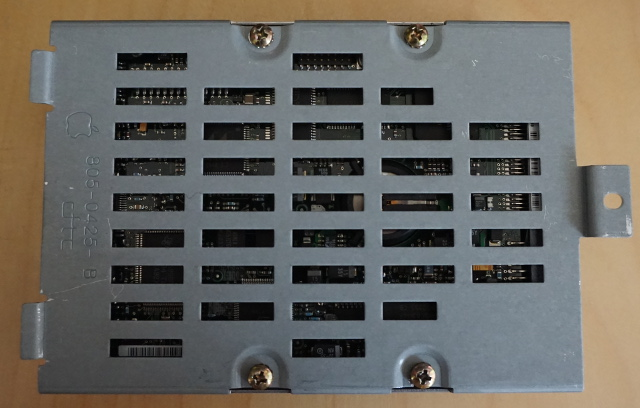5. Remove the HDD from the plate.

6. Affix the SCSI2SD device to the new adapter, and then to the original steel plate, using the standoffs and nuts: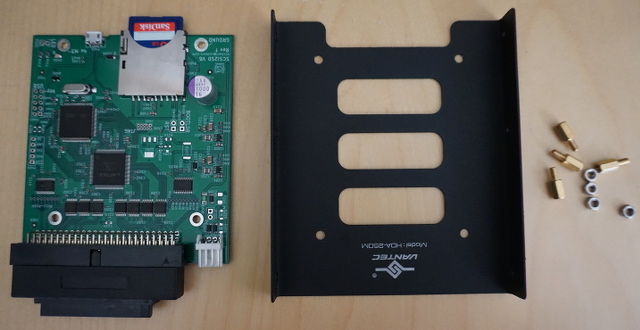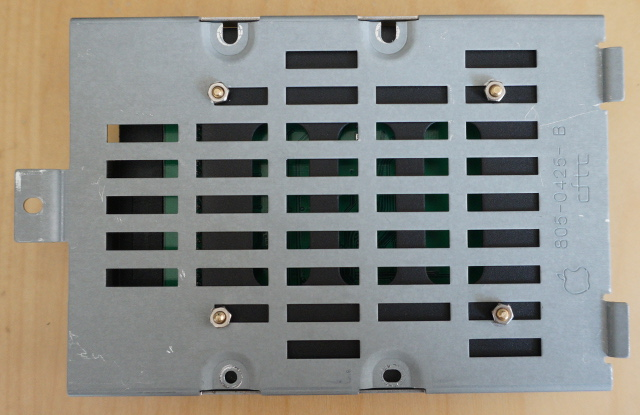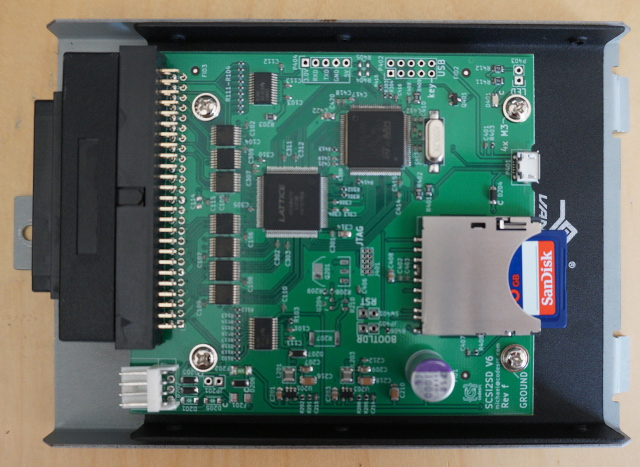Do not over-tension the nuts. (Use a tension wrench, if you have one, otherwise simply "know measure".)

7. Affix the plate, now containing SCSI2SD device, into the original nest, and connect the cabling, via the 50-to-68-pin adapter (for SCSI) and the Molex-to-floppy (for +5v):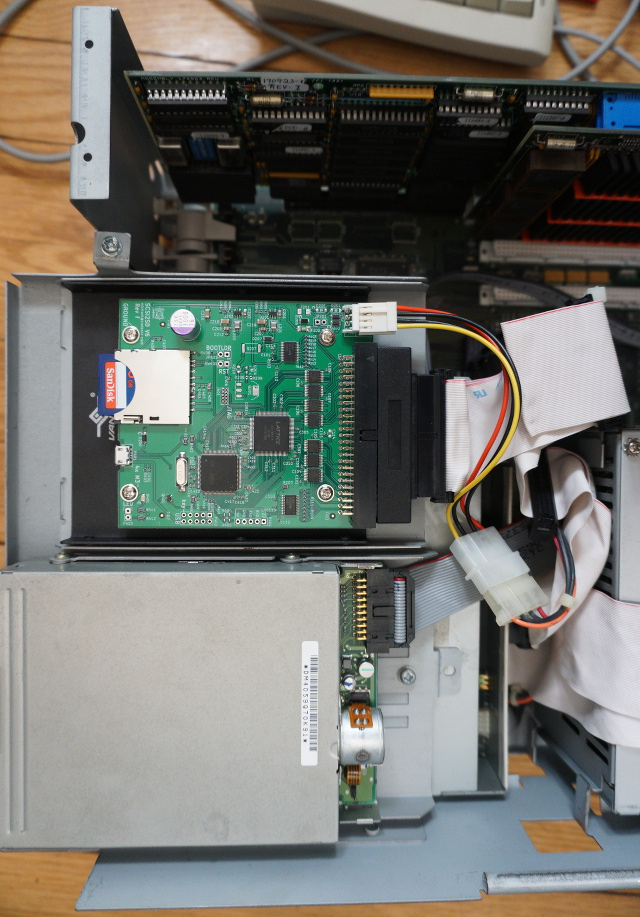8. Boot!

You can now back up and otherwise manipulate the contents of the MacIvory HDD by connecting the SCSI2SD device's USB jack to a Linux PC, or by simply removing the SD card.

~Fin~

## Symbolics "MacIvory": PCB Photographs.

This post concerns the "MacIvory" Model 3 Lisp Machine. It is of interest strictly to bolixologists.

Click on a photo to see detailed version.

The Ivory NuBus Board Set (i.e. the LispM itself, the Mac Quadra is otherwise ordinary):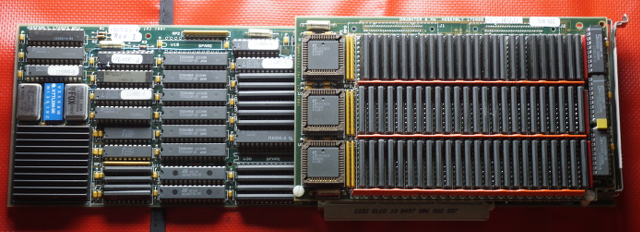"Ivory" NuBus board, component-side: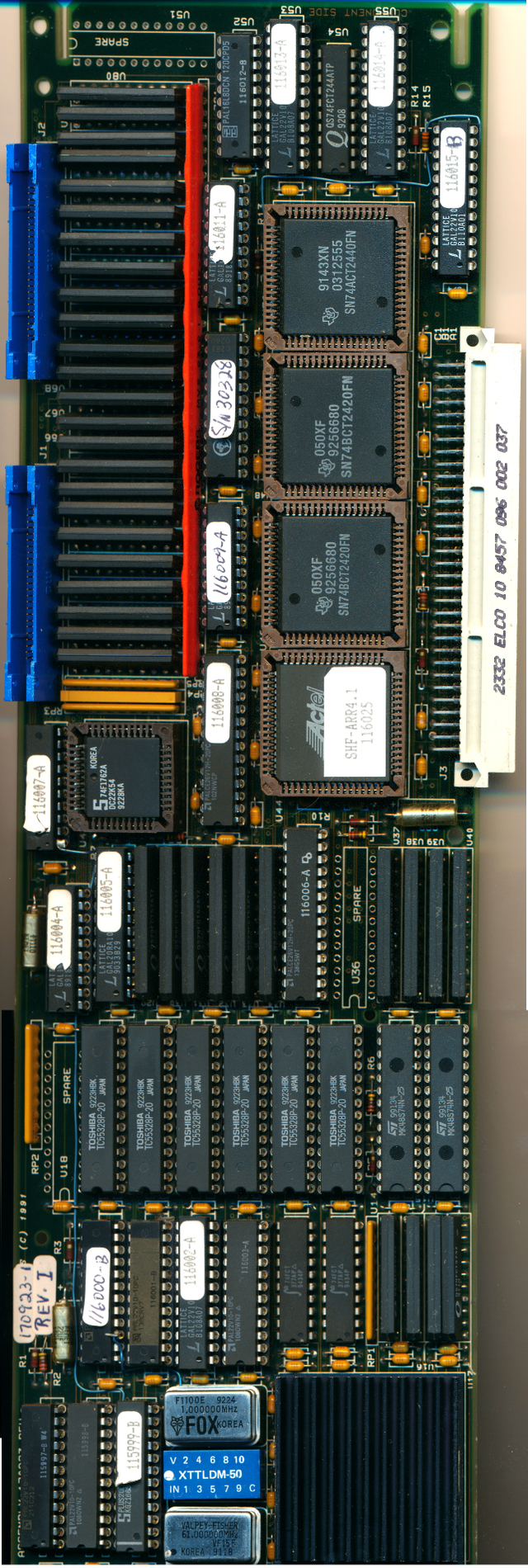## What's under the labels?

Bolix Label Part Notes
115999-B PLUS20L8 PAL, 14 inputs, 8 outputs, no registers
116000-B PAL22V10-10P PAL, 12 inputs, 10 macrocells, can have registers
116001-B PAL22V10-10P PAL, 12 inputs, 10 macrocells, can have registers
116004-A GAL16V8 GAL, can have registers
116007-A PAL16R8-7PC PAL, 8 inputs, 8 registers
116011-A GAL16V8 GAL, can have registers
S/N 30328 CY7C261-55PC 8K x 8 PROM, probably unit serial number
SHF-ARR4.1/116025 Actel A1010A-1 Antifuse-programmed CPLD, 1200 gates (equiv. of 12 period PALs)

## What are the vertical DIP ICs???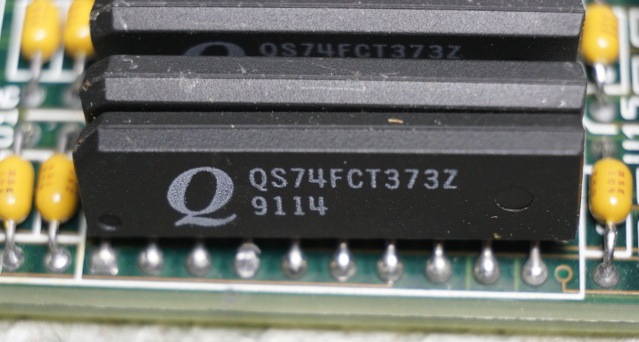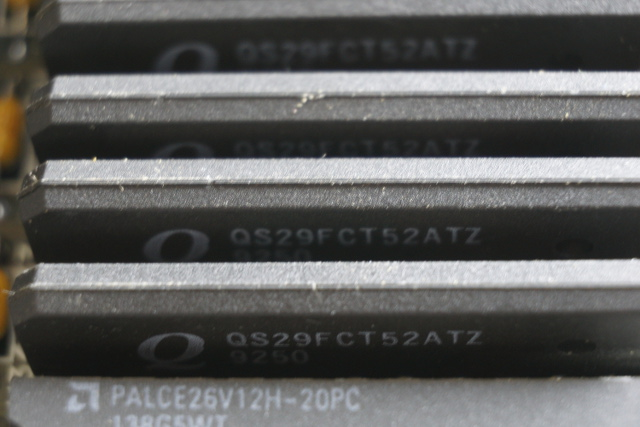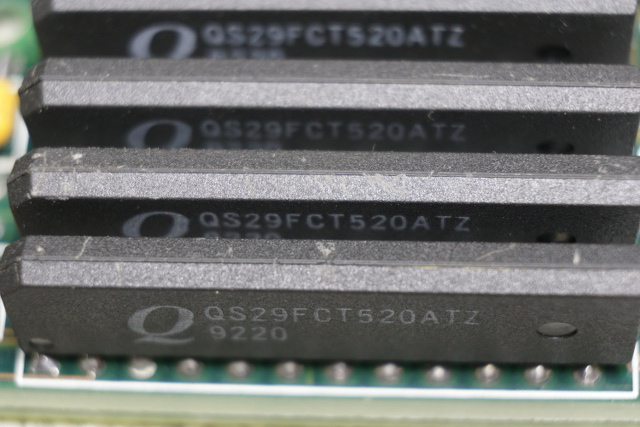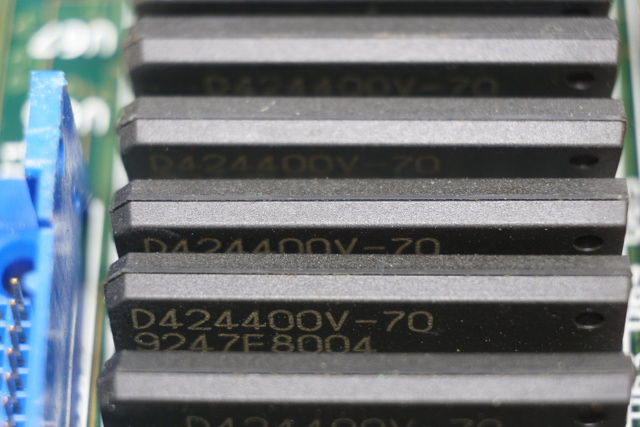"Ivory" NuBus board, bottom-side: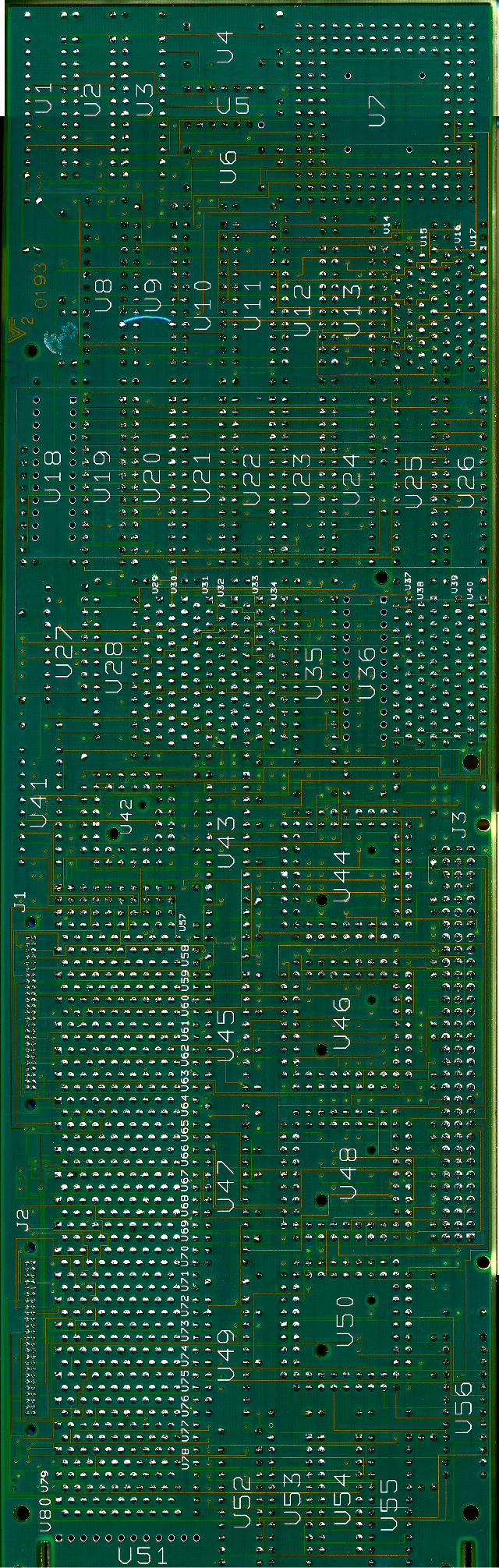"Ivory" RAM daughterboard, component-side: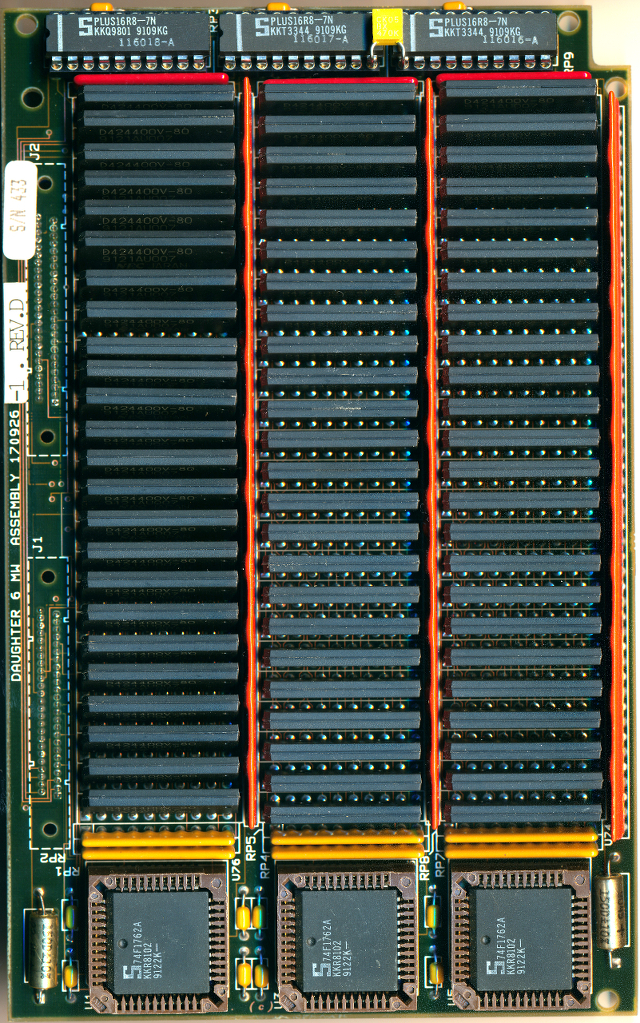## What are the vertical DIP ICs???"Ivory" RAM daughterboard, bottom-side: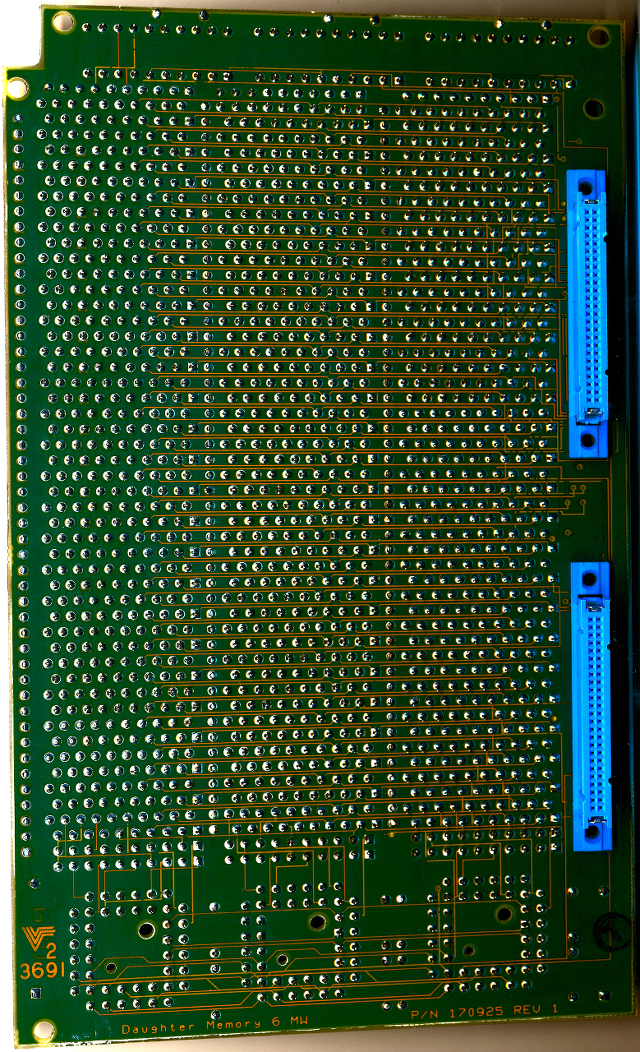Original 2400dpi scans of the boards (pre-photostitching) are available here:
1, 2, 3, 4, 5, 6 (WARNING: 200-300MB each!)

~To Be Continued~

## “Finite Field Arithmetic” vs MPI.

Let's compare the CPU cost of modular exponentiation performed on Chapter 14 FFA vs ye olde MPI.

V-press the MPI tree to mpi_second_cut.vpatch (or use diana_coman's cleaned-up variant, this should not affect the result of the test.)

Now, replace the test_mpi.c example I provided, with the following MPIistic implementation of the Ch.14 example test tape:

```#include "mpi.h" #include <stdlib.h> #include <stdio.h>     int main(int ac, char **av) { MPI base, exp, mod, res, known; int r, cmp;   r = secmem_init(1000); if (r==0) { fprintf(stderr, "eggog\n"); exit(1); }   base = mpi_alloc_secure(0); exp = mpi_alloc_secure(0); mod = mpi_alloc_secure(0); res = mpi_alloc_secure(0); known = mpi_alloc_secure(0);   mpi_fromstr(base, "0x09C98FCB9C40EC96BB9ECE3FD053D8468614D2C262A6ED7ACC613968CAF8A58FA725" "E113CCB16A000BF82170353B71789E23DD4620966EE23191C97F0290606CB7AEF750CF" "9EB6716BB474BA28DD3A615D3FC3D19812ABB2735676C7EBD505497A3080BF349F2833" "5439FC0567C150006CE796D9F4307B0A5C0617619C0F4C29CB496D164F09E363A8D535" "149B3485D4D0F0C8F2395CBC8E067910CDD9A0228AE6E2E37CA22F75EE91944C231899" "A1B340DB9968C6AB1EC7DBA911B0AF3CD3AD1AE83573A51A7DAC53E8FFCBF9D985BE9C" "C003409ADB8E068CBE71243C4A4EB678D169489FD0818B91C35F9A073DD2BC06173E13" "578481C1E98692B79C0A1CBB");   mpi_fromstr(exp, "0x513C8694309772EA44FEA976BE4DD5674F0A798D20385E1F18D71ACA6FD1680D9A09" "E9F075BEB7FE8A30911106618A4BC961CC0442E9BB8939D0CB249FEA16ECECB6762ADE" "FD1CD660842CDA6CF7EC2EF4C523E61E8F119A1357AF40DAE7E1ECE49F27C23728CD51" "86D197802AD03098ACFD8E058BD7F8340FFFB5E8ACB807D7B96D223FB0EC6D6E68C01C" "6293BC94B5BD370888C192F9C62C617B1598B8F19914C5F98DBB5B9D06936B5E97BDDE" "87FEB0192C9EB0A32D9F7B066D134FB5E9215FF2440340DC33568F5F4441A25F040D81" "CF584923A7DF28135F5F30282D344278E60D8DBCB0D36C8641057265406E31896C6BC1" "5DB82F0BDAFC5F456C9EB35F");   mpi_fromstr(mod, "0x1F8FF0E6C3FF3A40C18BBAB99D9DB19A6413C734507638D6F44B90848D7B4FE2E87E" "4D830B14F03E7FD87F47CCD4A715C51839952C5DB5B3F04E4C9633964881B761E763D7" "45B5DF2F8649418CB4B3DCA692535B862FC2C62F11DCEE2AB4191B25B35A6DEDC58547" "6DACF952A2F580C061F9DCD6C0CE4F08BF401379EC47CED1DE5A3C87EC98CE4DFFC70E" "B65A3C6700C5145107D3DD305BA1E0E0F6A957566A0452BE16E39CF4A17185644FBA8E" "D1CA79A3D6E28D29D936F2F953913EFBB94B7AF0CAA81CF3F5EF5C86E2F115424FEEEB" "D1DAD7FB371E27B2D727381F441ED5377E3DAB15BCF786E88582D6533AF673FA86047E" "BBC1667E6A12DD7102359052");     mpi_fromstr(known, "0x0FCB1DE435944F516B3F05D990C761985348076167AFCF56FEA05BE1DBA81286BA21" "39B0D10232577B79A4B9B872244E966EDC256678D1132B206D357D90F1F601B663DFD6" "2FE78449A0D1E4B7E7FC55C2B978282D37B70A6D8715451BC114282B3E9555E23612C2" "974974FA52ADDC6F2B030F0E98E6C5C6747C23A58FAE4C8730A37426B54EC604EA1EF1" "6F24B1FE8B190A993FC28A95960987D1768AC731DEC4E6B334C75B27589F0E99DAC4A7" "AC5CA9E7A014C2E0F05A72A18145A9B8958D0F26E179D3854E3C8CD0C3DD8E11DA318F" "9BE873175B1168580CEA42593FEC2795FDBDBC349D10B76118E646AA8EE5C2295452C2" "E78DC26528F7C4A4D2F90E21");   mpi_powm(res, base, exp, mod);   mpi_free(base); mpi_free(exp); mpi_free(mod);   cmp = mpi_cmp(res, known);   if (cmp == 0) { fprintf(stdout, "OK\n"); } else { fprintf(stdout, "SAD\n"); }   mpi_free(res); mpi_free(known);   fprintf(stdout, "\n");   secmem_term();   return 0; }```

Build it, and fire the test:

`\$ time ./koch`

... on my test iron, will produce the output:

```OK   real 0m0.639s user 0m0.636s sys 0m0.001s```

Now, let's feed the equivalent problem to Ch. 14 FFA:

```.09C98FCB9C40EC96BB9ECE3FD053D8468614D2C262A6ED7ACC613968CAF8A58F A725E113CCB16A000BF82170353B71789E23DD4620966EE23191C97F0290606CB 7AEF750CF9EB6716BB474BA28DD3A615D3FC3D19812ABB2735676C7EBD505497A 3080BF349F28335439FC0567C150006CE796D9F4307B0A5C0617619C0F4C29CB4 96D164F09E363A8D535149B3485D4D0F0C8F2395CBC8E067910CDD9A0228AE6E2 E37CA22F75EE91944C231899A1B340DB9968C6AB1EC7DBA911B0AF3CD3AD1AE83 573A51A7DAC53E8FFCBF9D985BE9CC003409ADB8E068CBE71243C4A4EB678D169 489FD0818B91C35F9A073DD2BC06173E13578481C1E98692B79C0A1CBB   .513C8694309772EA44FEA976BE4DD5674F0A798D20385E1F18D71ACA6FD1680D 9A09E9F075BEB7FE8A30911106618A4BC961CC0442E9BB8939D0CB249FEA16ECE CB6762ADEFD1CD660842CDA6CF7EC2EF4C523E61E8F119A1357AF40DAE7E1ECE4 9F27C23728CD5186D197802AD03098ACFD8E058BD7F8340FFFB5E8ACB807D7B96 D223FB0EC6D6E68C01C6293BC94B5BD370888C192F9C62C617B1598B8F19914C5 F98DBB5B9D06936B5E97BDDE87FEB0192C9EB0A32D9F7B066D134FB5E9215FF24 40340DC33568F5F4441A25F040D81CF584923A7DF28135F5F30282D344278E60D 8DBCB0D36C8641057265406E31896C6BC15DB82F0BDAFC5F456C9EB35F   .1F8FF0E6C3FF3A40C18BBAB99D9DB19A6413C734507638D6F44B90848D7B4FE2 E87E4D830B14F03E7FD87F47CCD4A715C51839952C5DB5B3F04E4C9633964881B 761E763D745B5DF2F8649418CB4B3DCA692535B862FC2C62F11DCEE2AB4191B25 B35A6DEDC585476DACF952A2F580C061F9DCD6C0CE4F08BF401379EC47CED1DE5 A3C87EC98CE4DFFC70EB65A3C6700C5145107D3DD305BA1E0E0F6A957566A0452 BE16E39CF4A17185644FBA8ED1CA79A3D6E28D29D936F2F953913EFBB94B7AF0C AA81CF3F5EF5C86E2F115424FEEEBD1DAD7FB371E27B2D727381F441ED5377E3D AB15BCF786E88582D6533AF673FA86047EBBC1667E6A12DD7102359052   MX   .0FCB1DE435944F516B3F05D990C761985348076167AFCF56FEA05BE1DBA81286 BA2139B0D10232577B79A4B9B872244E966EDC256678D1132B206D357D90F1F60 1B663DFD62FE78449A0D1E4B7E7FC55C2B978282D37B70A6D8715451BC114282B 3E9555E23612C2974974FA52ADDC6F2B030F0E98E6C5C6747C23A58FAE4C8730A 37426B54EC604EA1EF16F24B1FE8B190A993FC28A95960987D1768AC731DEC4E6 B334C75B27589F0E99DAC4A7AC5CA9E7A014C2E0F05A72A18145A9B8958D0F26E 179D3854E3C8CD0C3DD8E11DA318F9BE873175B1168580CEA42593FEC2795FDBD BC349D10B76118E646AA8EE5C2295452C2E78DC26528F7C4A4D2F90E21   ={(do nothing if ok)}{[SAD ]}_```

`\$ time cat 2048.tape | ./bin/ffa_calc 2048 32`

... and we get, on same test iron:

```real 0m0.280s user 0m0.277s sys 0m0.002s```

Turns out, Koch's pile of shit, despite eschewing constant time arithmetic, and being implemented in Overflowandcrashlang... loses the footrace, when given a full-width modular exponentiation (i.e. one where it cannot cheat by skipping over leading zeroes.)

## “Finite Field Arithmetic.” Chapter 14B: Barrett's Modular Reduction. (Part 2 of 2.)

This article is part of a series of hands-on tutorials introducing FFA, or the Finite Field Arithmetic library. FFA differs from the typical "Open Sores" abomination, in that -- rather than trusting the author blindly with their lives -- prospective users are expected to read and fully understand every single line. In exactly the same manner that you would understand and pack your own parachute. The reader will assemble and test a working FFA with his own hands, and at the same time grasp the purpose of each moving part therein.

You will need:

Add the above vpatches and seals to your V-set, and press to ffa_ch14_barrett.kv.vpatch.

You should end up with the same directory structure as previously.

Now compile ffacalc:

```cd ffacalc gprbuild```

But do not run it quite yet.

First, the mail bag!

Reader diana_coman recently observed that the OF_in parameter taken by certain procedures in FZ_Shift is not checked for validity, and -- if abused by being given an oversized (i.e. wider than the given shiftness) value -- could result in a garbage output.

Her observation is entirely correct. And ideally, the range of OF_in would be constrained via a precondition. Unfortunately, Ada does not permit the use of preconditions in combination with inlining; and FZ_Shift routines are invoked in several costly inner loops, and absolutely must be subject to inlining. Therefore, it is impractical to actually verify the bit-width of OF_in on every invocation. It is, however, the case that these procedures are defined strictly for internal use in FFA, and hence do not constitute a danger to the operator. After giving the matter some thought, I took diana_coman's suggestion and added comments to warn the reader of the potential rake he could step on if he were to insist on making direct use of FFA's internal shift routines.

I will take this opportunity to remind the reader that FFA is designed to be "safe if used as prescribed": if it is invoked via the provided external interface, the promised semantics are guaranteed to apply. The only prohibited operations are ones which over- or under-run the FFACalc stack, demand a division by zero, or attempt to violate other FFACalc rules. (These will bring the program to an orderly stop, and warn the operator.) All other actions will produce arithmetically-correct outputs for the given inputs. However it is impractical on extant iron to make this guarantee for each of the internal components taken separately!

This is why we want sane iron, with inexpensive bounds-checking instructions! But we do not have it yet. Hence, the reader who wishes to make use of FFA internals for some custom purpose of his own, must proceed with extreme caution.

Reader diana_coman also observed that Get_Argument in FFACalc's command line handler:

``` procedure Get_Argument(Number : in Natural; Result : out String);```

... can be turned into the stricter type:

``` procedure Get_Argument(Number : in Natural; Result : out CmdLineArg);```

Thank you for these nitpicks, diana_coman! And for reading and signing Chapters 3, 4, 5, and 6:

Reader mircea_popescu observed that Chapter 13's FZ_Measure can be slightly simplified, where:

`Index := W_Mux(Index + 1, Index, W_ZeroP(W));`

... can be safely turned into the equivalent:

`Index := Index + W_NZeroP(W);`

I have included the change in this Chapter. Thank you, reader mircea_popescu !

Now, let's eat the meat of this Chapter.

We'll start with a very minor extension of FFACalc. A Version command has been introduced:

Op Description # Ins # Outs Notes
V Put the FFACalc and FFA version numbers on the stack. 0 2 Kelvin Versioning is in use.

The implementation of this command is quite straightforward:

``` -- Put the FFACalc Program Version on the stack, -- followed by FFA Program Version. when 'V' => Push; Push; -- FFACalc Version: FFA_FZ_Clear(Stack(SP - 1)); FFA_FZ_Set_Head(Stack(SP - 1), Word(FFACalc_K_Version)); -- FFA Version: FFA_FZ_Clear(Stack(SP)); FFA_FZ_Set_Head(Stack(SP), Word(FFA_K_Version));```

```package Version is   pragma Pure;   ---------------------------------------------- -- Current 'deg. Kelvin' Version of FFACalc -- ---------------------------------------------- FFACalc_K_Version : constant Natural := 255; ----------------------------------------------   end Version;```

``` -- ...   ---------------------------------------------------------------------------- --- Current 'deg. Kelvin' Version of FFA ----------------------------------------------------------------------------   FFA_K_Version : constant Natural := 255;   -- ...```

The effect: FFACalc and FFA now have independent "Degrees Kelvin" versions -- i.e. they are to decrement by one upon every published revision to each respective program. Observe that this constitutes a promise to the reader: no more than 255 changes to either FFACalc or FFA are to be published after this Chapter. In the quite unlikely event where a change is found to be required after a Kelvin version reaches zero degrees, it is expected that the program is to be renamed, Vtronically-reground, and some very pointed questions posed to the maintainer!

Now, let's proceed to the originally-planned subject of Chapter 14B: the Ada implementation of Barrett's Modular Reduction.Don't even think about proceeding further into this Chapter if you have not fully read and understood the two previous chapters:

Stop now and go back, study! Lest you become a danger to yourself and others.

We will now discuss the Ada implementation of the Algorithm 2 given in Chapter 14A. Please print the Algorithm and the physical bounds proof and refer to these while reading this Chapter.

```package FZ_Barr is   pragma Pure;   -- Precomputed data for Barrett's Modular Reduction type Barretoid(ZXMLength : Indices; BarretoidLength : Indices) is record ZXM : FZ(1 .. ZXMLength); -- Zero-Extended Modulus J : FZBit_Index; -- Jm B : FZ(1 .. BarretoidLength); -- The Barrettoid itself ZSlide : FZBit_Index; -- Amount to slide Z Degenerate : WBool; -- Is it degenerate case? end record;     -- Prepare the precomputed Barrettoid corresponding to a given Modulus procedure FZ_Make_Barrettoid(Modulus : in FZ; Result : out Barretoid) with Pre => Result.B'Length = 2 * Modulus'Length and Result.ZXM'Length = Modulus'Length + 1;     -- Reduce N using the given precomputed Barrettoid. procedure FZ_Barrett_Reduce(X : in FZ; Bar : in Barretoid; XReduced : in out FZ); pragma Inline_Always(FZ_Barrett_Reduce);   end FZ_Barr;```

In every instance of the pre-computed Barrettoid data structure, we will keep everything which is required for Barrett's Modular Reduction by a given modulus. In particular, we will retain ZXM: a zero-extended (for convenient use in steps 6 and 8) copy of the modulus itself; the parameter JM; the Barrettoid proper, BM; ZSlide, the number of bits we must right-shift Z by to compute ZS; and, finally, the degeneracy indicator, i.e. DM.

Unsurprisingly, a Barrettoid is computed from a given modulus with the procedure FZ_Make_Barrettoid. All Barrettoids -- like other FFA data -- will exist as stack-allocations. (Heapism in any form whatsoever is forever banned in FFA.) And the only use of a Barrettoid is to compute Barrett's Modular Reduction, using FZ_Barrett_Reduce. We will review both procedures in detail, below.

Here is how we create a Barrettoid:

``` -- Prepare the precomputed Barrettoid corresponding to a given Modulus procedure FZ_Make_Barrettoid(Modulus : in FZ; Result : out Barretoid) is   -- Length of Modulus and Remainder Lm : constant Indices := Modulus'Length;   -- Remainder register, starts as zero Remainder : FZ(1 .. Lm) := (others => 0);   -- Length of Quotient, with an extra Word for top bit (if Degenerate) Lq : constant Indices := (2 * Lm) + 1;   -- Valid indices into Quotient, using the above subtype Quotient_Index is Word_Index range 1 .. Lq;   -- The Quotient we need, i.e. 2^(2 * ModulusBitness) / Modulus Quotient : FZ(Quotient_Index);   -- Permissible 'cuts' for the Slice operation subtype Divisor_Cuts is Word_Index range 2 .. Lm;   -- Current bit of Pseudo-Dividend; high bit is 1, all others 0 Pb : WBool := 1;   -- Performs Restoring Division on a given segment procedure Slice(Index : Quotient_Index; Cut : Divisor_Cuts; Bits : Positive) is begin   declare   -- Borrow, from comparator C : WBool;   -- Left-Shift Overflow LsO : WBool;   -- Current cut of Remainder register Rs : FZ renames Remainder(1 .. Cut);   -- Current cut of Divisor Ds : FZ renames Modulus(1 .. Cut);   -- Current Word of Quotient being made, starting from the highest W : Word := 0;   -- Current bit of Quotient (inverted) nQb : WBool;   begin   -- For each bit in the current Pseudo-Dividend Word: for b in 1 .. Bits loop   -- Advance Rs, shifting in the current Pseudo-Dividend bit: FZ_ShiftLeft_O_I(N => Rs, ShiftedN => Rs, Count => 1, OF_In => Pb, -- Current Pseudo-Dividend bit Overflow => LsO);   -- Subtract Divisor-Cut from R-Cut; Underflow goes into C: FZ_Sub(X => Rs, Y => Ds, Difference => Rs, Underflow => C);   -- Negation of current Quotient bit nQb := C and W_Not(LsO);   -- If C=1, the subtraction underflowed, and we must undo it: FZ_Add_Gated(X => Rs, Y => Ds, Sum => Rs, Gate => nQb);   -- Save the bit of Quotient that we have found: W := Shift_Left(W, 1) or (1 - nQb); -- i.e. inverse of nQb   end loop;   -- We made a complete Word of the Quotient; save it: Quotient(Quotient'Last + 1 - Index) := W; -- Indexed from end   end;   end Slice; pragma Inline_Always(Slice);   -- Measure of the Modulus ModulusMeasure : constant FZBit_Index := FZ_Measure(Modulus);   begin   -- First, process the high Word of the Pseudo-Dividend: Slice(1, 2, 1); -- ... it has just one bit: a 1 at the bottom position   -- Once we ate the top 1 bit of Pseudo-Dividend, rest of its bits are 0 Pb := 0;   -- Process the Modulus-sized segment below the top Word: for i in 2 .. Lm - 1 loop   Slice(i, i + 1, Bitness); -- stay ahead by a word to handle carry   end loop;   -- Process remaining Words: for i in Lm .. Lq loop   Slice(i, Lm, Bitness);   end loop;   -- Record the Quotient (i.e. the Barrettoid proper, Bm) Result.B := Quotient(Result.B'Range);   -- Record whether we have the Degenerate Case (1 iff Modulus = 1) Result.Degenerate := W_NZeroP(Quotient(Lq));   -- Record a copy of the Modulus, extended with zero Word: Result.ZXM(Modulus'Range) := Modulus; Result.ZXM(Result.ZXM'Last) := 0;   -- Record the parameter Jm: Result.J := ModulusMeasure - 1;   -- Wm - Jm Result.ZSlide := FZBit_Index(Bitness * Modulus'Length) - ModulusMeasure + 1;   end FZ_Make_Barrettoid;```

The process may seem complicated, but it is merely a specialized form of FZ_Mod. With the difference that we are interested in the quotient, rather the remainder, and also wish to compute certain additional parameters corresponding to the given modulus.

Recall that a Barrettoid BM for a given modulus M, was defined as the quantity ⌊2k / M⌋. In FZ_Make_Barrettoid, we compute it via Knuth's division. Afterwards we record: the modulus itself; the quotient; whether the given modulus corresponds to the degenerate case M = 1; the parameter JM; and the parameter ZSlide. After this, the contents of the Barrettoid can be used to perform modular reduction modulo M in constant time.

And now, let's show exactly how:

``` -- Reduce X using the given precomputed Barrettoid. procedure FZ_Barrett_Reduce(X : in FZ; Bar : in Barretoid; XReduced : in out FZ) is   -- Wordness of X, the quantity being reduced Xl : constant Indices := X'Length;   -- Wordness of XReduced (result), one-half of Xl, and same as of Modulus Ml : constant Indices := XReduced'Length; -- i.e. # of Words in Wm.   -- The Modulus we will reduce X by Modulus : FZ renames Bar.ZXM(1 .. Ml);   -- Shifted X Xs : FZ(X'Range);   -- Z := Xs * Bm (has twice the length of X) Z : FZ(1 .. 2 * Xl);   -- Upper 3Wm-bit segment of Z that gets shifted to form Zs ZHi : FZ renames Z(Ml + 1 .. Z'Last );   -- Middle 2Wm-bit segment of Z, that gets multiplied by M to form Q Zs : FZ renames Z(Z'First + Ml .. Z'Last - Ml );   -- Sub-terms of the Zs * M multiplication: ZsLo : FZ renames Zs(Zs'First .. Zs'Last - Ml ); ZsHi : FZ renames Zs(Zs'First + Ml .. Zs'Last ); ZsHiM : FZ(1 .. Ml);   -- Q := Modulus * Zs, i.e. floor(X / M)*M + E*M Q : FZ(1 .. Xl); QHi : FZ renames Q(Q'First + Ml .. Q'Last );   -- R is made one Word longer than Modulus (see proof re: why) Rl : constant Indices := Ml + 1;   -- Reduction estimate, overshot by 0, 1, or 2 multiple of Modulus R : FZ(1 .. Rl);   -- Scratch for the outputs of the gated subtractions S : FZ(1 .. Rl);   -- Borrow from the gated subtractions C : WBool;   -- Barring cosmic ray, no underflow can take place in (4) and (5) NoCarry : WZeroOrDie := 0;   begin   -- Result is initially zero (and will stay zero if Modulus = 1) FZ_Clear(XReduced);   -- (1) Ns := X >> Jm FZ_Quiet_ShiftRight(N => X, ShiftedN => Xs, Count => Bar.J);   -- (2) Z := X * Bm FZ_Multiply_Unbuffered(X => Bar.B, Y => Xs, XY => Z);   -- (3) Zs := Z >> 2Wm - Jm (already thrown lower Wm, so only Wm - Jm now) FZ_Quiet_ShiftRight(N => ZHi, ShiftedN => ZHi, Count => Bar.ZSlide);   -- (4) Q := Zs * M (this is split into three operations, see below)   -- ... first, Q := ZsLo * M, FZ_Multiply_Unbuffered(ZsLo, Modulus, Q);   -- ... then, compute ZsHiM := ZsHi * M, FZ_Low_Multiply_Unbuffered(ZsHi, Modulus, ZsHiM);   -- ... finally, add ZsHiM to upper half of Q. FZ_Add_D(X => QHi, Y => ZsHiM, Overflow => NoCarry);   -- (5) R := X - Q (we only need Rl-sized segments of X and Q here) FZ_Sub(X => X(1 .. Rl), Y => Q(1 .. Rl), Difference => R, Underflow => NoCarry);   -- (6) S1 := R - M, C1 := Borrow (1st gated subtraction of Modulus) FZ_Sub(X => R, Y => Bar.ZXM, Difference => S, Underflow => C);   -- (7) R := {C1=0 -> S1, C1=1 -> R} FZ_Mux(X => S, Y => R, Result => R, Sel => C);   -- (8) S2 := R - M, C2 := Borrow (2nd gated subtraction of Modulus) FZ_Sub(X => R, Y => Bar.ZXM, Difference => S, Underflow => C);   -- (9) R := {C2=0 -> S2, C2=1 -> R} FZ_Mux(X => S, Y => R, Result => R, Sel => C);   -- (10) RFinal := {DM=0 -> R, DM=1 -> 0} (handle the degenerate case) FZ_Mux(X => R(1 .. Ml), Y => XReduced, Result => XReduced, Sel => Bar.Degenerate); -- If Modulus = 1, then XReduced is 0.   end FZ_Barrett_Reduce;```

Notice anything unfamiliar? The astute reader will observe that the above is an exact implementation of the process described in Chapter 14A-Bis; the only new subcomponent is the FZ_Low_Multiply_Unbuffered routine used in Step 4. So let's learn what it's made of.

But first, review the elementary multiplication equivalence from Chapter 10:

 XLo XHi × YLo YHi = XLoYLo + XLoYHi + XHiYLo + XHiYHi = XY

Suppose, however, that we were only interested in calculating the bottom half of XY. We can then write the following schematic instead:

 XLoYLo + (XLoYHi)Lo + (XHiYLo)Lo = XYLo

Observe that this method is exactly analogous to the mechanism used in Chapter 9's Mul_Word, where we find the lower half of a Word × Word multiplication by:

``` -- ........   -- XL * YL LL : constant Word := Mul_HalfWord_Iron(XL, YL);   -- XL * YH LH : constant Word := Mul_HalfWord_Iron(XL, YH);   -- XH * YL HL : constant Word := Mul_HalfWord_Iron(XH, YL);   -- ........   begin   -- Get the bottom half of the Product: XY_LW := LL + Shift_Left(LH + HL, HalfBitness);   -- ........```

So let's now see how this works for FZ rather than Word:

```-- "Low Multiplication" computes only the bottom half of the product XY. -- Presently, it is used solely in Barrett's Modular Reduction.   package body FZ_LoMul is   -- Low-Only Comba's multiplier. (CAUTION: UNBUFFERED) procedure FZ_Low_Mul_Comba(X : in FZ; Y : in FZ; XY : out FZ) is   -- Words in each multiplicand (and also in the half-product) L : constant Word_Index := X'Length;   -- 3-word Accumulator A2, A1, A0 : Word := 0;   begin   -- Compute the lower half of the Product, which is all we want: for N in 0 .. L - 1 loop   -- Compute the Nth (indexed from zero) column of the Half-Product declare   -- The outputs of a Word multiplication Lo, Hi : Word;   -- Carry for the Accumulator addition C : WBool;   -- Sum for Accumulator addition Sum : Word;   begin   -- For lower half of XY, will go from 0 to N -- For upper half of XY, will go from N - L + 1 to L - 1 for j in 0 .. N loop   -- Hi:Lo := j-th Word of X * (N - j)-th Word of Y Mul_Word(X(X'First + j), Y(Y'First - j + N), Lo, Hi);   -- Now add Hi:Lo into the Accumulator:   -- A0 += Lo; C := Carry Sum := A0 + Lo; C := W_Carry(A0, Lo, Sum); A0 := Sum;   -- A1 += Hi + C; C := Carry Sum := A1 + Hi + C; C := W_Carry(A1, Hi, Sum); A1 := Sum;   -- A2 += A2 + C A2 := A2 + C;   end loop;   -- We now have the Nth (indexed from zero) word of XY XY(XY'First + N) := A0;   -- Right-Shift the Accumulator by one Word width: A0 := A1; A1 := A2; A2 := 0; end;   end loop;   end FZ_Low_Mul_Comba;     -- Low-Only Multiplier. (CAUTION: UNBUFFERED) procedure Low_Mul(X : in FZ; Y : in FZ; XY : out FZ) is   -- L is the wordness of a multiplicand. Guaranteed to be a power of two. L : constant Word_Count := X'Length;   -- K is HALF of the length of a multiplicand. K : constant Word_Index := L / 2;   -- A 'KSeg' is the same length as HALF of a multiplicand. subtype KSeg is FZ(1 .. K);   -- The two K-sized variables of the half-product equation: LH, HL : KSeg;   -- Bottom and Top K-sized halves of the multiplicand X. XLo : KSeg renames X( X'First .. X'Last - K ); XHi : KSeg renames X( X'First + K .. X'Last );   -- Bottom and Top K-sized halves of the multiplicand Y. YLo : KSeg renames Y( Y'First .. Y'Last - K ); YHi : KSeg renames Y( Y'First + K .. Y'Last );   -- Top K-sized half of the half-product XY. XYHi : KSeg renames XY( XY'First + K .. XY'Last );   -- Carry from individual term additions. C : WBool; pragma Unreferenced(C);   begin   -- Recurse to FULL-width multiplication: XY := XLo * YLo FZ_Multiply_Unbuffered(XLo, YLo, XY);   -- Recurse to HALF-width multiplication: LH := XLo * YHi FZ_Low_Multiply_Unbuffered(XLo, YHi, LH);   -- Recurse to HALF-width multiplication: HL := XHi * YLo FZ_Low_Multiply_Unbuffered(XHi, YLo, HL);   -- XY += 2^(K * Bitness) * LH FZ_Add_D(X => XYHi, Y => LH, Overflow => C);   -- XY += 2^(K * Bitness) * HL FZ_Add_D(X => XYHi, Y => HL, Overflow => C);   end Low_Mul; -- CAUTION: Inlining prohibited for Low_Mul !     -- Low-Only Multiplier. (CAUTION: UNBUFFERED) procedure FZ_Low_Multiply_Unbuffered(X : in FZ; Y : in FZ; XY : out FZ) is   -- The length of either multiplicand L : constant Word_Count := X'Length;   begin   if L < = Low_Mul_Thresh then   -- Base case: FZ_Low_Mul_Comba(X, Y, XY);   else   -- Recursive case: Low_Mul(X, Y, XY);   end if;   end FZ_Low_Multiply_Unbuffered;```

FZ_Low_Mul_Comba, of course, is merely a cut-down Comba from Chapter 9; while the recursion is analogous to the one in Chapter 10's Karatsuba and Chapter 12's Square Karatsuba.

Now, let's see where Barrett's Reduction is put to use. You will recall the conclusion of Chapter 12B, where we discussed the fact that the use of Knuth's division for modular reduction is quite expensive, and constitutes the bulk of the cost of modular exponentiation as presented in Chapters 6 through 13. And now, at last, we can write a fast modular reducer -- one that uses Barrett's method instead of Knuth's division:

``` -- (Barrettronic) Modular Exponent: Result := Base^Exponent mod Modulus procedure FZ_Mod_Exp(Base : in FZ; Exponent : in FZ; Modulus : in FZ; Result : out FZ) is   -- Double-width scratch buffer for the modular operations D : FZ(1 .. Base'Length * 2);   -- Working register for the squaring; initially is copy of Base B : FZ(Base'Range) := Base;   -- Register for the Mux operation T : FZ(Result'Range);   -- Buffer register for the Result R : FZ(Result'Range);   -- Space for Barrettoid Bar : Barretoid(ZXMLength => Modulus'Length + 1, BarretoidLength => 2 * B'Length);   begin   -- First, pre-compute the Barretoid for the given Modulus: FZ_Make_Barrettoid(Modulus => Modulus, Result => Bar);   -- Result := 1 WBool_To_FZ(1, R);   -- For each Word of the Exponent: for i in Exponent'Range loop   declare   -- The current Word of the Exponent Wi : Word := Exponent(i);   begin   -- For each bit of Wi: for j in 1 .. Bitness loop   -- T := Result * B mod Modulus FZ_Multiply_Unbuffered(X => R, Y => B, XY => D); FZ_Barrett_Reduce(X => D, Bar => Bar, XReduced => T);   -- Sel is the current bit of Exponent; -- When Sel=0 -> Result := Result; -- When Sel=1 -> Result := T FZ_Mux(X => R, Y => T, Result => R, Sel => Wi and 1);   -- Advance to the next bit of Wi (i.e. next bit of Exponent) Wi := Shift_Right(Wi, 1);   -- B := B^2 mod Modulus FZ_Square_Unbuffered(X => B, XX => D); FZ_Barrett_Reduce(X => D, Bar => Bar, XReduced => B);   end loop;   end;   end loop;   -- Output the Result: Result := R;   end FZ_Mod_Exp;```

Quite straightforward: we precompute the Barrettoid, and use it for all of the necessary modular multiplications and squarings modulo the given modulus.

The reader may be curious regarding how to properly test this program. And so I will invite him to download a complete package of test tapes, generated using a RNG:

Each modular exponentiation test tape was mechanically-produced on Chapter 13 FFA, and contains a series of modular exponentiations, each followed by an equality comparison with the expected result. E.g., a 2048-bit test:

```.09C98FCB9C40EC96BB9ECE3FD053D8468614D2C262A6ED7ACC613968CAF8A58F A725E113CCB16A000BF82170353B71789E23DD4620966EE23191C97F0290606CB 7AEF750CF9EB6716BB474BA28DD3A615D3FC3D19812ABB2735676C7EBD505497A 3080BF349F28335439FC0567C150006CE796D9F4307B0A5C0617619C0F4C29CB4 96D164F09E363A8D535149B3485D4D0F0C8F2395CBC8E067910CDD9A0228AE6E2 E37CA22F75EE91944C231899A1B340DB9968C6AB1EC7DBA911B0AF3CD3AD1AE83 573A51A7DAC53E8FFCBF9D985BE9CC003409ADB8E068CBE71243C4A4EB678D169 489FD0818B91C35F9A073DD2BC06173E13578481C1E98692B79C0A1CBB   .513C8694309772EA44FEA976BE4DD5674F0A798D20385E1F18D71ACA6FD1680D 9A09E9F075BEB7FE8A30911106618A4BC961CC0442E9BB8939D0CB249FEA16ECE CB6762ADEFD1CD660842CDA6CF7EC2EF4C523E61E8F119A1357AF40DAE7E1ECE4 9F27C23728CD5186D197802AD03098ACFD8E058BD7F8340FFFB5E8ACB807D7B96 D223FB0EC6D6E68C01C6293BC94B5BD370888C192F9C62C617B1598B8F19914C5 F98DBB5B9D06936B5E97BDDE87FEB0192C9EB0A32D9F7B066D134FB5E9215FF24 40340DC33568F5F4441A25F040D81CF584923A7DF28135F5F30282D344278E60D 8DBCB0D36C8641057265406E31896C6BC15DB82F0BDAFC5F456C9EB35F   .1F8FF0E6C3FF3A40C18BBAB99D9DB19A6413C734507638D6F44B90848D7B4FE2 E87E4D830B14F03E7FD87F47CCD4A715C51839952C5DB5B3F04E4C9633964881B 761E763D745B5DF2F8649418CB4B3DCA692535B862FC2C62F11DCEE2AB4191B25 B35A6DEDC585476DACF952A2F580C061F9DCD6C0CE4F08BF401379EC47CED1DE5 A3C87EC98CE4DFFC70EB65A3C6700C5145107D3DD305BA1E0E0F6A957566A0452 BE16E39CF4A17185644FBA8ED1CA79A3D6E28D29D936F2F953913EFBB94B7AF0C AA81CF3F5EF5C86E2F115424FEEEBD1DAD7FB371E27B2D727381F441ED5377E3D AB15BCF786E88582D6533AF673FA86047EBBC1667E6A12DD7102359052   MX   .0FCB1DE435944F516B3F05D990C761985348076167AFCF56FEA05BE1DBA81286 BA2139B0D10232577B79A4B9B872244E966EDC256678D1132B206D357D90F1F60 1B663DFD62FE78449A0D1E4B7E7FC55C2B978282D37B70A6D8715451BC114282B 3E9555E23612C2974974FA52ADDC6F2B030F0E98E6C5C6747C23A58FAE4C8730A 37426B54EC604EA1EF16F24B1FE8B190A993FC28A95960987D1768AC731DEC4E6 B334C75B27589F0E99DAC4A7AC5CA9E7A014C2E0F05A72A18145A9B8958D0F26E 179D3854E3C8CD0C3DD8E11DA318F9BE873175B1168580CEA42593FEC2795FDBD BC349D10B76118E646AA8EE5C2295452C2E78DC26528F7C4A4D2F90E21   ={(do nothing if ok)}{[SAD ]}_```

One invokes the tapes as follows, e.g. for the 1024-bit 10,000 shot tape:

`\$ time cat 10k_shots_1024bit_ffa_unif_rnd.tape | ./bin/ffa_calc 1024 32`

... and if successful (i.e. all outputs are correct) it will emit only the output of the unix "time" command; e.g. on my test iron:

``` real 7m24.751s user 7m24.081s sys 0m0.290s```

Now, the reader has probably read Dijkstra and recalls that "testing can reveal the presence of bugs, but never their absence." So why bother? The answer is, it is necessary to test your iron.

The test tapes in the signed TAR come in two variants, slid (where there are randomly-sized stretches of leading zeroes in the arguments to modular exponentiation) and uniform -- where there are not. You can use the test tapes as a litmus of whether your iron provides a constant-time iron multiplier and a constant-time barrel shifter. If you find that the "slid" tapes reliably execute faster on your machine than the "unif" tapes of the same respective FFA width, you have sad iron and must enable the workarounds (i.e. Mul_HalfWord_Soft and/or HaveBarrelShifter := False.)

Now let's find out what we actually achieve by using Barrett's Reduction: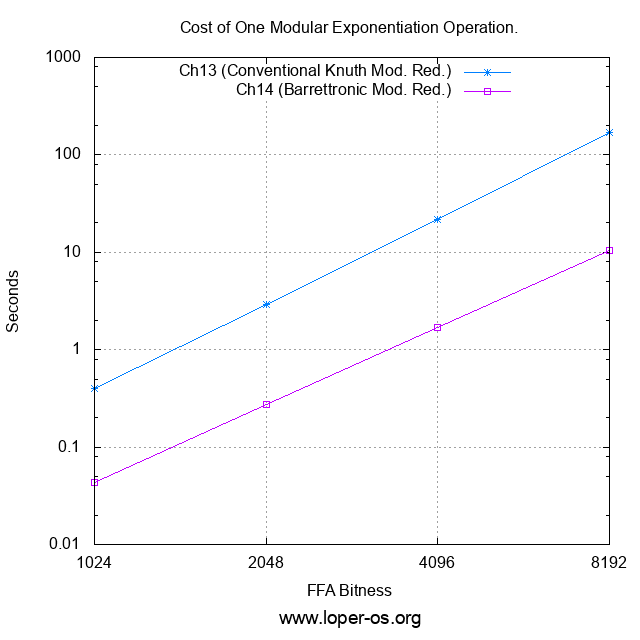Or, for those who prefer the raw numbers to the logarithmic plot,

Cost of one modular exponentiation operation (sec):
FFA Bitness Ch.13 (Conventional Knuth Mod. Red.) Ch.14 (Barrettronic Mod. Red.)
1024 0.395 0.043
2048 2.895 0.276
4096 21.895 1.703
8192 169.394 10.400

It would appear that "the game was worth the candles" -- we now have (AFAIK: the first and only presently-published...) fully constant-time Barrettron. And it is one that (with reasonable effort on the reader's part) fits-in-head.

In the next chapter, 15, we will begin to assemble the necessary ingredients for the generation of cryptographic primes. Please stay tuned!

~To be continued!~

## “Finite Field Arithmetic.” Chapter 14A-Bis: Barrett's Modular Reduction. (Physical Bounds Proof.)

This article is part of a series of hands-on tutorials introducing FFA, or the Finite Field Arithmetic library. FFA differs from the typical "Open Sores" abomination, in that -- rather than trusting the author blindly with their lives -- prospective users are expected to read and fully understand every single line. In exactly the same manner that you would understand and pack your own parachute. The reader will assemble and test a working FFA with his own hands, and at the same time grasp the purpose of each moving part therein.

You will need:

• All of the materials from Chapters 1 - 14A
• There is no vpatch in Chapter 14A-Bis.

Given the trickiness of Barrett's Reduction under the constraints of FFA, and the fact that we want to hard-guarantee, as adults do, the exact amount of physical space required to hold the intermediate results of the computation, it is necessary to extend the proof of Chapter 14A with a physical bounds proof -- before we can dare to look at the corresponding program code.

We will illuminate said proof with "electrical" illustrations, in the style of Chapter 12A.

Please print out and refer to Algorithm 2 from Chapter 14A when working through this Chapter.

First, three lemmas:

Lemma 1:
For any integers A ≥ 0, B > 0, C = ⌊A / B⌋, Measure(C) ≤ Measure(A) - Measure(B) + 1.

Lemma 2:

For any integers A ≥ 0, B ≥ 0, C = A × B, Measure(C) ≤ Measure(A) + Measure(B).

Lemma 3:

For any integers A ≥ 0, B ≥ 0, C = A + B, Measure(C) ≤ Max(Measure(A), Measure(B)) + 1.

If the correctness of these is not obvious, dear reader, stop reading and prove them. Right now.

We will use ⇠dashed arrows⇢ to refer to the variant bitness bounds of hypothetical zero and nonzero segments of registers, for the purpose of min/max width analysis of the intermediate results in our computation. ←Solid arrows→ will refer to invariant bounds (i.e. the physical sizes of particular registers.)

First, let's describe the physical space occupied by the quantities which must exist prior to performing Barrett's Reduction of a given X.

We begin with the modulus: 0 < M < 2WM. It lives in a WM-bit register:

 ←WM→ M

Now let's picture, strictly for illustrative purposes, an M which is a bit less than "half-full":

 ←WM→ 0 M ⇠JM+1⇢

Recall that we picked the concrete value of JM (0 ≤ JM < WM) to equal one less than the measure of M, i.e.,
JM = Measure(M) - 1.

Observe that this value is a secret (just like M and X) and therefore it is forbidden in FFA to branch on its bits, index memory by it, etc. The only mechanical thing we will do with it is to shift certain registers (via a constant-time method) by it.

Now, let's describe the Barrettoid BM corresponding to a given M.

By Lemma 1, the Measure of 0 < BM < 2KM will always be less than or equal to:

(2WM + 1) - Measure(M) + 1 = 2WM - JM.

And so, BM will look like:

 ←2WM→ 0 BM ⇠JM⇢ ⇠2WM - JM⇢

Now, of course, we also need the dividend:

0 ≤ X < 2KM is the integer to be reduced modulo M, and its register has twice the bitness of M:

 X ←2WM→

Now, let's describe the space required to carry out Barrett's Reduction itself, where in the end we obtain X mod M.

The first of the two shifts in the algorithm, where we obtain the term ⌊X / 2j:

1: Xs = X >> JM

 ←2WM→ 0 XS ⇠JM⇢ ⇠2WM - JM⇢

The first of the two multiplications. This one is 2WM × 2WM-sized, and it will give us the intermediate result:
⌊X / 2j⌋⌊2k / M⌋.
The second multiplicand is our pre-computed Barrettoid for the current M. And so:

2: Z = Xs × BM

By Lemma 2:

 ←2WM→ 0 XS ⇠JM⇢ ⇠2WM - JM⇢ ←2WM→ × 0 BM ⇠JM⇢ ⇠2WM - JM⇢ ←4WM→ = 0 Z ⇠2JM⇢ ⇠4WM - 2JM⇢

The second of the two shifts. This one gets us the entire Barrett approximation of the quotient, i.e. ⌊X / M⌋ + E :

 ⌊2k / M⌋⌊X / 2j⌋ ——————————————— 2k - j

3: Zs := Z >> 2WM - JM

 ←4WM→ 0 ZS ⇠2WM + JM⇢ ⇠2WM - JM⇢

Observe that we do not need to spend the cost of shifting the whole of Z in order to obtain ZS, given as the shift will always be of at least WM+1-bits (since 0 ≤ JM < WM). Ergo, the lower WM bits of Z are always discarded in this process, and can be simply ignored:

 ←4WM→ 0 Z ⇠2JM⇢ ⇠4WM - 2JM⇢
 ←4WM→ = ZHi ZMid ZLo ←WM→ ←2WM→ ←WM→

... so it actually suffices to right-shift only the 3WM-bit segment consisting of ZHi and ZMid, by WM - JM bits, and afterwards to take the ZMid-wide lower segment of the output as the resulting ZS.

ZS always fits inside a 2WM-bit register:

 ←2WM→ 0 ZS ⇠JM⇢ ⇠2WM - JM⇢

Here, we have something a little more interesting. We have an asymmetric (2WM × WM-sized) multiplication, where we want to multiply ZS, the Barrett approximation of the quotient, by the modulus M:

4: Q := Zs × M

 ←2WM→ 0 ZS ⇠JM⇢ ⇠2WM - JM⇢ ←WM→ × 0 M ⇠JM+1⇢←3WM→ = 0 Q ←WM→ ←2WM→

By Lemma 2, a 2WM-bit × WM-bit product in the general case must occupy 3WM bits; while, looking closer, it would appear that, given as we have multiplied the (2WM - JM)-bit contents of ZS by the JM+1-bit contents of M, we could end up with a 2WM+1-bit result...

However, in Chapter 14A we proved that:

 ⌊2k / M⌋⌊X / 2j⌋ X mod M = X - M × ——————————————— - E × M 2k - j

And so we know that Q ≤ X, and therefore, regardless of the value of JM, the term Q will always fit inside 2WM bits.

 ←2WM→ Q ←2WM→ X

If the "secret" of this "miracle" eludes you, please go back to Chapter 14A and walk the proof, until it fits-in-head.

Now, we find the initial estimate of X mod M:

5: R := X - Q

 ←2WM→ X ←2WM→ - Q ←2WM→ = R

But do we actually need to perform a 2WM-bit subtraction here? It turns out that we don't, because R is at most W+2-bit. We know this because we have proven that at most two subtractions of M -- a WM-bit quantity -- will be required after this step to produce the final, correct X mod M, a WM-bit quantity. Therefore, what we actually need to do instead of the above, is:

 ←2WM→ Ignore X ←WM - L→ ←WM + L→ ←2WM→ - Ignore Q ←WM - L→ ←WM + L→ = R ←WM + L→

L here is the bitness of our machine Word.

And by Lemma 3, we know that each of the following two gated subtractions can remove a maximum of one bit from the length of R; and from the Chapter 14A proof, we know that the final result will be of the same maximal bitness (i.e. will fit in the same size of FFA register) as the modulus M.

Now, we will do the first of our two gated subtractions. We will subtract a WM + L zero-extended (on the senior end, by one machine word) M from R, to obtain a difference S1 and a "borrow", C1.

6: S1 := R - M, C1 := Borrow

 ←WM + L→ R ←WM + L→ - 0 M ←L→ ←WM→ ←WM + L→ = S1 C1 ← Borrow

Now, the "gating" of that subtraction:

7: R := {C1=0 → S1, C1=1 → R}

 ←WM + L→ ←WM + L→ S1 ⇒Mux(C1)⇐ R ↳C1=0⇘ ⇓ ⇙C1=1↲ R ←WM + L→

That is, if the subtraction in step 6 did not underflow, as indicated by C1 = 0, then we know that R was greater than or equal to M prior to step 6, and we then keep S1 (the result of the subtraction) and assign it back to R. On the other hand, if it did underflow, as indicated by C1 = 1, then we discard S1, and R stays as it was. Note that this process is to be carried out in constant time, via FZ_Mux.

Now, the second of the two gated subtractions. Exactly like in Step 6:

8: S2 := R - M, C2 := Borrow

 ←WM + L→ R ←WM + L→ - 0 M ←L→ ←WM→ ←WM + L→ = S2 C2 ← Borrow

And, finally, the "gating" of the second (and last) subtraction from Step 8:

9: R := {C2=0 → S2, C2=1 → R}

 ←WM + L→ ←WM + L→ S2 ⇒Mux(C2)⇐ R ↳C2=0⇘ ⇓ ⇙C2=1↲ R ←WM + L→

Observe that we are not yet finished with R. The final result R = X mod M could still be one of two possible things:

• If M ≠ 1, the final result will be found in the bottom WM bits of R.
• If M = 1, then we had the degenerate case, and everything we did in steps 1-9 was simply to occupy constant-time, and has no bearing on the answer to the calculation of X mod M; the final result in this case is to be zero.

Ergo, we need one last Mux:

10: RFinal := {DM=0 → R, DM=1 → 0}

First, observe that:

 ←WM + L→ R = 0 RF ←L→ ←WM→

The two gated subtractions will at this point have zeroed any of the L upper bits of R that may have been previously non-zero.

And so, all that remains to do now, is:

 ←WM→ ←WM→ RF ⇒Mux(DM)⇐ 0 ↳DM=0⇘ ⇓ ⇙DM=1↲ R = X mod M ←WM→

...and that's it, we have R = X mod M, as was proven already in Chapter 14A.

In the next Chapter, 14B, we will finally walk through the Ada program which implements this Barrettron. Stay tuned!